# Working with equations

Equations are the mathematical basis for simulating the behaviour of a model. All node-type elements with the exception of file parameters, and all flows and squirts, must have equations for the model to run.

Before its equation is entered, a model element is displayed in red. It is not possible to run the simulation whilst any model elements are still red. If you make a change to the model that causes a component's equation to become invalid, for instance deleting an influence going to that component, it will be displayed in red to indicate that its equation must be edited before the model can run. Simile has an equation language that allows great power and flexibility in specifying expressions, reading data from tables, or sketching semi-quantitative relationships by hand.

## How to enter equations

There are two ways to enter equations for calculating model element values. Either use:

In either case, a helpful error message will appear if you enter an equation that cannot be used by Simile.

## How to write equations

The following topics provide detailed reference information on the parts that make up a complex expression, and on the mathematical functions available, both built-in and user-supplied. See:

## When is the equation evaluated?

• For compartments and population initializers (creation), the equation defines the initial value, and is applied when the model is first built, when it is reset, or when the submodel instance containing the compartment or population comes into existence (for instance, if it is itself in a population submodel).

• For rule-based state variables, the 'On reset...' equation is evaluated whenever a compartment's would be. The other equations are evaluated when their corresponding events occur.

• For events and squirts, the equation is only evaluated when they occur.

• For other components, the equation defines their value at any time while the model is running.

## Working with variables and data types

Further information on variables and data types useful in understanding Simile.

In: Contents

# The equation bar

The equation bar offers a quick and simple way to enter equations for any elements on the model diagram.

• Select the pointer tool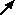• Click on the element whose equation you wish to edit.

The name of the element is placed on the left hand side of the equation bar.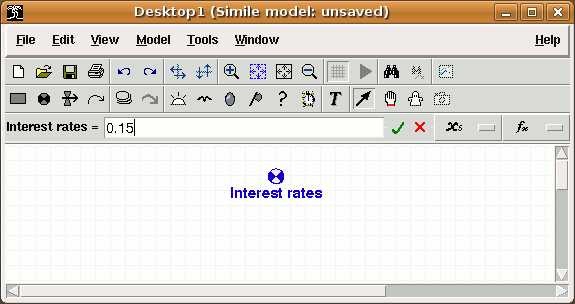• Click on the equation bar edit box to enter a value or an expression for the element.
• Click on the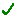button, or hit return, to set the equation.
• Click on the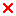button if you make a mistake. It restores the previous entry.

Elements which are calculated from other elements, i.e. are at the end of one or more influence arrows, have the local names of those elements listed in a drop-down list under the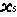button.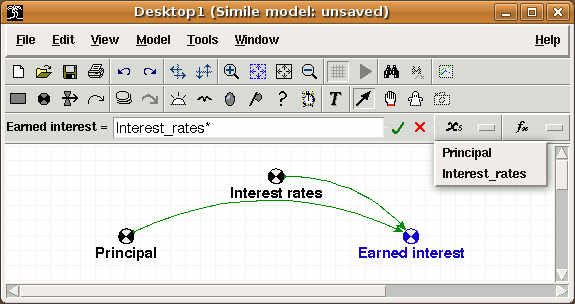• Click on any name listed in the drop-down box to enter it into the equation. The name is added at the position of the cursor in the equation text.

A selection of the most-commonly used functions are listed in a menu cascade under the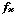button. The top level of this cascade also contains an entry labelled "Enum. type constants", for adding the names and members of any enumerated types defined in the hierarchy containing the selected component. This also allows access to the "boolean" unit type and its values.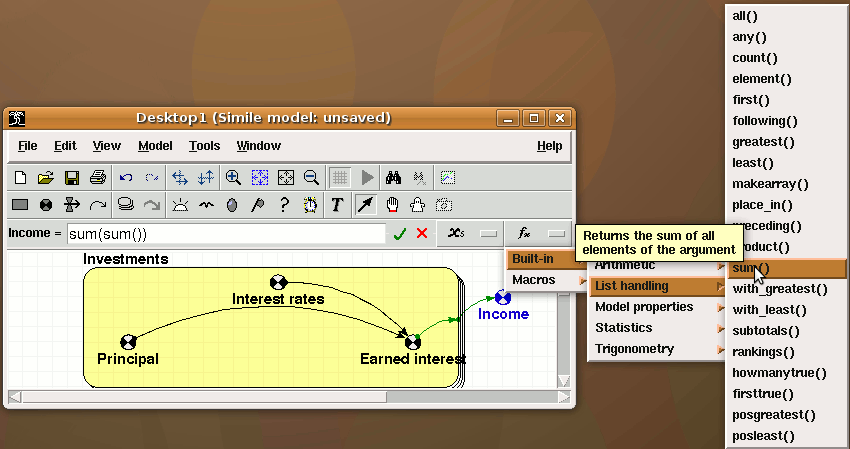• Click on any function that appears in the menu cascade to enter it into the equation. The function is added at the position of the cursor in the equation text, and the cursor is moved to inside the parentheses after the function, so you can immediately add its arguments. One more useful thing: if you select part of the text in the equation bar before adding a function from the menus, the function is added with its parentheses around the selection, so the selection becomes the argument.

## Auto-complete feature

Typing in the equation bar activates the auto-complete feature. If the first few characters typed match the start of any entry in the cascade menu described above, i.e., valid input parameters, function names or enumerated type names or members, the remaining text in the shortest of those possible matches will be inserted into the text and highlit. The cursor moves to the end of the highlit text. The modeller can then do one of the following:

• Hit the left or right arrow, return or tab to keep the inserted text. If a function name has been completed, the text includes the pair of parentheses that will enclose its arguments, so hitting left-arrow will keep the completion and position the cursor within the parentheses ready to add the arguments.
• Hit the up arrow; this will select alternative completions from the same initial characters.
• Continue to type; the completion text will be ignored. Completions will continue to appear as long as the typed characters still match some menu entries.

## Re-use previously entered equation

The entry field in the equation bar has a drop-down list containing equations previously entered in the current editing session. The list can be accessed by hitting down-arrow while editing the equation, or by clicking the down-arrow button to the right of the entry field. This is useful if you need to add similar equations for a lot of components (though you should think about using a single component in a multiple-instance submodel instead!) Also if you leave the equation bar and the equation produces an error message, you need to click on the component again to get back into the equation bar after which the equation you entered will be gone -- you can get it back from the drop-down list to edit it and fix the problem.

# Equation dialogue window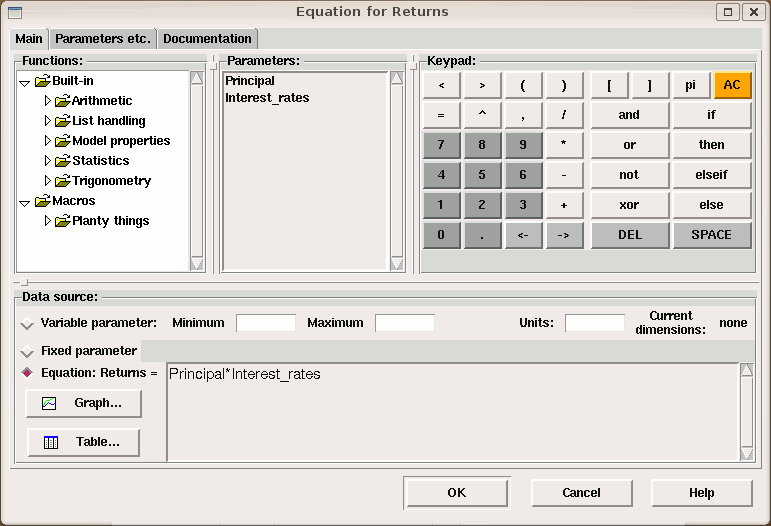The equation dialogue window is used to identify the data source used to calculate a value for a model element, as well as to set various other properties of the model element. The same dialogue window is used for all the node-type elements, e.g.,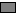compartments,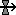flows and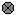variables, as well as the control symbols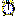iterations and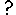conditions, and the population symbols. For the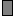state symbol, the dialogue appears a little differently because an equation must be entered for each trigger event that influences the state. (Note that three other model elements,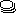submodels,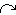influence arrows, and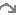role arrows each have their own dialogue box).

To open the equation dialogue window:

• Select the pointer tool
• Doubleclick on the component whose equation you wish to edit.

Alternatively:

• Right-click on the component to be edited
• Select 'Properties...' from the context menu.

The equation dialogue window consists of a notebook with three tabs. The first of these, labelled 'Main', is initially topmost, and contains the following panels which help you create the equation for your component:

## Functions:

This panel displays a tree diagram containing all Simile's built-in functions, plus any user-defined functions that have been added on your system. They are grouped into categories, and have popups giving a brief description of each function. Doubleclicking on a function name will insert the function into the equation at the cursor position, and leave the cursor between the parentheses ready for argument expressions to be entered there if needed. If you have selected part of the equation text, the function is added with the selected text inside its parentheses.

## Parameters:

The parameters in an equation are the values of the components that are linked to its own component by influence arrows. The parameters panel will show all the names associated with the parameters that have been connected. These are usually the same as the names of the components they come from, but may be different to avoid duplication, or in the case where a role arrow is being used to select only certain values from the component.

You can double-click on the parameter names to insert them into your equation, or hover over them to display a popup showing the location of their source and any roles that have been used to select their values. The next tab includes a panel that allows you to change the local names of the parameters.

This panel contains an array of buttons which insert text into whichever entry field has the cursor. They include buttons for moving the cursor back and forth, deleting a character and deleting all the text.

The second to right button on the top row inserts customizable text. By default it is set to µ since this character might otherwise be hard to find (it represents the micro- prefix for units) but you can set it to other characters or strings using the entry under the Edit tab in the Preferences dialogue window. Another useful setting for this might be π, which is interpreted as its trigonometrical value, or e if you are entering a lot of numbers in scientific notation.

## Data source:

These three radio buttons set the data source to one of the following options:

1. Variable parameter/Time series event: the value for the model element is set from the equation when the model is built, and can be changed during the simulation. The value can be set using a scenario file, and displayed and set through a user-operated slider control. This option is only relevant if the model element is at the beginning of a chain of influences. It cannot be used to alter the value of a model element that is calculated from the values of other model elements. The minimum and maximum values that the parameter can accept must be entered in the neighbouring text boxes. For a full explanation see the help sections Variable parameters and Time series events, and for a full explanation of working with sliders, see the help section Sliders.

1. Fixed parameter: the value for the model element is set when the model is built and does not change. The value can be set using a scenario file. This option is only relevant if the model element is at the beginning of a chain of influences. It cannot be used to alter the value of a model element that is calculated from the values of other model elements. An equation can be entered, but it is only used for setting the array dimensions of the parameter. i.e., its value is never used. For a full explanation of working with fixed parameters, see the help section on Fixed parameters an for a full explanation of working with scenario files, see the help section Working with external data. Not available for events.

2. Limit event: This option is only available for events. If it is chosen, the equation is not the value of the event but is used to determine when the event occurs, by detecting when its value reaches one of the supplied bounds.

1. Equation: the value for the model element is calculated using the expression given in the text box. The expression is first evaluated when the model is built, and subsequently re-evaluated at each time step. For a full explanation of equations, see the help section Components of an equation. In brief, equations consist of functions operating on the values of the other model elements that influence this one. A list of functions is provided. Double-click on any of the function names to insert it into the equation text box. A list of the indices of the model element is also given. One index is listed for each level of nesting of the submodel(s) containing the model element. Double-click on any of the index names to insert the corresponding index() function into the equation text. Finally, a list of the other model elements (if any) that influence this one is presented. Double-click on any of the model element names to insert the name into the equation text.

### Explicitly defined functons:

An equation can include one sketch graph or table function, which can be used exactly like any other function. These require extra data to be supplied, so they are added by clicking on buttons. If you just type graph() or table() into your equation, you will get an error message complaining that no data has been supplied to specify the behaviour of the function.

• Graph: This button enables the user to sketch a graph for a mathematical relationship. The relationship is sketched in a window opened up by clicking on this button. Having done this, a reference to a function graph() is automatically added to the expression in the Equation box. This function is evaluated at run time by referring to the graph sketched by the model developer. See the help entry for the graph function for more information.

• Table: This button enables a user to specify a tabulated relationship. The tabulated values are held in a data file, and clicking on this button enables you to browse through your file system for the data file, which can be in a variety of formats, and then choose the particular column of data you require from this file. Having done this, a reference to a function table() is automatically added to the expression in the Equation box. The function is evaluated at run time by doing a table look-up on the values provided. See the help entry for the table function for more information.

The data source panel also contains entry fields for minimum and maximum values and for units. Min/max values have various uses in simulation but do not actually constrain the value of the component. They set the scaling of display tools to fit the likely values that will be taken by the model components. Since the I/O tools have access to these values it would be possible to create an I/O tool that would display a warning if a value goes outside its minimum/maximum range. Minimum and maximum must be entered for variable parameters in order to set the range of values for their slider controls when the model runs. For compartments, the model execution can be set up to produce a message if the compartment's value goes outside the specified minimum or maximum. For limit events, they set the values which the equation must reach to trigger an occurrence.

The units entry field can usually be ignored, because a default units value will be generated from the equation and inserted there automatically. It only needs editing if you want a non-default unit (e.g., a real value for an integer constant) or if you want to specify the physical units of a quantity, such as metres per second.

The panel also shows the current array dimensions of the model element. The dimensions depend on the equation and the dimensions of any parameters, so they are not editable. For instance the result of sum() will have one less dimension than its argument, while the result of makearray() will have one more.

The following panels are to be found on the second tab, "Parameters, etc..."

## Indices:

If your component is inside a multiple instance submodel you can use the index(n) function to get the identity of the submodel instance currently being evaluated. However if you are inside many such submodels, multidimensional submodels, or particularly association submodels where you can also use index(n) to get the indices of the current base model instances, it can be confusing working out which nesting level you want. So this panel contains a list of all the available index arguments with popups explaining which index each one gives. Again, doubleclicking them inserts them into the equation text.

## Influences

If the component has influence arrows connected to it from other components in the diagram, these will be listed in the Influences panel, along with their units and array dimensions if any. Doubleclicking on an influence's name will insert it into the equation text at the cursor position.

Hover the mouse over the local name of a model element listed in the influences list box to see the full path name of the model element. The local name is automatically composed from the full path name by replacing characters that have other meanings in equations (such as spaces, parentheses and carriage returns) with underscores. If you would prefer to use a different local name to refer to the full path name, the local name can be edited. Click on the name in the list box to edit it. The name you enter will only be used in this equation, which must use this name.

In the case where role arrows are used to select only a certain set of values from a component in another submodel, the default local name will also include the apropriate role arrow's caption. For the case of two role arrows between the same pair of submodels, influences between the submodels will get two local names each, one for the values associated with each role.

The local name will be enclosed in square or curly brackets if it refers to an array or list (see below), with nested brackets for nested sructures. These make the equation more readable, and you must keep them when editing the local name.

The 'units' field can also be edited, to set the units that will be used to convert the physical quantity of the incoming parameter into a number which will be used in the equation. See the section working with physical units for a full explanation of how this field is used.

The 'dimensions' field cannot be edited. This tells you the array dimensions of the value that this parameter supplies. Normally this will be the dimensions of the source component itself multiplied by those of any submodels the influence comes out of, though special rules apply for population symbols (creation and immigration behave as if outside their submodels) and those taking part in roles (normally you get a list, but going backwards along an 'exclusive' role you get a single value).

## Documentation

Documentation appears on a separate tab in the dialogue box. You can enter a short title for the component and/or a longer description. For components with complex equations you can also put comments directly into the equation text by enclosing them in /* ... */ symbols.

# Working with equations : Understanding error messages

## Don't panic!

If you try to set an equation for a component that Simile would not be able to use to generate a value, an error message will appear in a dialogue, something like this: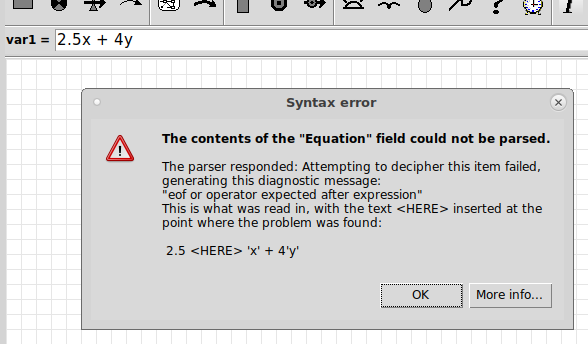The message is generated by Simile in an attempt to help you find out what is wrong with the equation and how to put it right.

### Syntax errors

There are two basic kinds of error messages. The first is the 'syntax error', an example of which is shown above. This is produced when the equation does not conform to the syntax, or grammar, of the equation language. Often the problem is the result of a typo, but it can also occur when the modeller uses a different idiom for writing an equation than the one Simile understands. For instance, in the above equation, the modeller has written '2.5x' to mean 'multiply x by 2.5', whereas Simile does not recognize this notation -- you need to include a multiplication symbol, as in '2.5*x'.

Simile uses the parser built into its Prolog engine to decode equations, but this takes a slightly different syntax than Simile's equation language, so the text is preprocessed first to make it compatible. In this case, single-quotes have been inserted around the variable names 'x' and 'y'. The parser reports the position at which it found the problem, and the error message shows the preprocessed text with <HERE> inserted at that position. It is usually clear from this what the problem was in the original equation.

Note that only the first syntax error is picked up -- the parser gives up after finding one. So just changing '2.5x' to '2.5*x' in this example will result in another syntax error, because '4y' also needs to be changed to '4*y'.

### Context errors

These are raised if the grammatical structure of the equation makes sense, but there are components that do not correspond to values, operators or functions in Simile, or functions are being used in a type of component in which they make no sense. Each kind of error has its own message, and the explanation should be sufficient to pinpoint the problem.

If you got the error message after clicking 'OK' in the equation dialogue, or hitting Newline or clicking 'tick' in the equation bar, then after you dismiss the message you will be back editing the equation, and you can try to fix the problem. If you get it after leaving the equation bar, you will have to click on the component again and use the pull-down menu to get the equation back into the bar before editing it.

The error message also has a 'More info...' button, although all the info is in fact displayed in the original message. The 'More info...' dialogue does have selectable text that can be copied and pasted for reporting, and a 'Help' button that brings you to this page.

# Components of an equation

Expressions can consist of one or more of these components: numerical constants, symbolic names, mathematical operators, functions, and conditional expressions.

## Numerical constants

Numerical constants are any numeric values, consisting of the digits 0-9, with an optional decimal point and a leading minus sign (for negative values). A leading 0 is optional for numbers less than 1.0. Standard scientific notation is provided (e.g. 0.12345E3 for 123.45), or you can enter very small or very large numbers by multiplying a value by 10 raised to a power, e.g. 0.12345*10^11 or 0.12345*10^-11. See also the section on Arrays and lists for details of how to enter arrays of numerical constants.

## Symbolic names

A symbolic name represents the value of one of the variables, compartments or flows that influences the element in question. Each time the value of the expression is calculated, the current value of the influencing element is used.

The only symbolic names for model quantities that you can enter into an expression are those that influence the element in question. These are listed by clicking on thebutton on the equation bar, and in the "Parameter" column of the equation dialogue box. These must be entered exactly as shown, using the same punctuation (note especially the _ underscore symbols), and using the same case. The easiest way to do this, is to double-click on the name in the "Parameter" column.

Note that the symbolic name entered into the expression is the local name. This can be different from the name displayed on the model diagram, either to make the expression easier to read, or to avoid using certain illegal characters.

## Mathematical operators

You can use the following mathematical operators:

 + e.g. 15+7 (evaluates to 22) - e.g. 15-7 (evaluates to 8) * e.g. 15*7 (evaluates to 105) / e.g. 15/7 (evaluates to 2.1428…) // integer division e.g. 15//7 (evaluates to 2) % integer modulo e.g. 94%22 (evaluates to 6) ^ raise to a power e.g. 15^2 (evaluates to 225)

## Functions

Simile provides a large number of functions that you can use in expressions already built-in, and the facility to extend this list.

## Conditional expressions

Equations can include conditional expressions. These enable a complex expression to be constructed from a number of sub-expressions, with the conditional elements being used to select between one expression or another. Standard Boolean operators are available for constructing conditional expressions.

## Intermediate variables

The equation dialogue box can be used to enter one or more assignments, before the expression that returns the element's value. These intermediate variables can be used to simplify complex expressions.

# Boolean expressions

Boolean expressions return only "true" or "false". Boolean expressions are commonly used in conditional expressions to decide between two or more alternative subexpressions, but a Simile variable can have a boolean value if its whole equation is a boolean expression; indeed, condition and alarm components have to have boolean values.

## Constants

The expressions "true" and "false" may be used as Boolean constants in expressions. Note that quotation marks must be used around the words. This is because these constants are implemented as built-in enumerated types.

## Mathematical comparisons

A simple condition consists of a direct comparison between two values, using the relational operators

 > greater than X > Y X is greater than Y < less than X < Y X is less than Y >= greater than or equal to X >= Y X is greater than or equal to Y <= less than or equal to X <= Y X is less than or equal to Y == equal to X == Y X is equal to Y != not equal to X != Y X is not equal to Y

Thus, the following are legal examples:

• 5 > 3 though it's hard to see the point of this, since this is always true
• height <= 30 where the variable "height" is an influencing variable

Each of the two terms, on the left and right of the comparison, can themselves be arbitrary mathematical expressions. Thus, the following are also legal:

• 5 > 3*2 (result is false, since 5 < 6)
• 0.1*height <= 30
• k*z+2 < 17*h

## Logical comparisons

Four Boolean operators can be used in expressions. There are "and", "or", "xor" and "not". The following table shows the two alternative forms of these operators, which can also written in symbolic form. Note also that a comma "," is accepted as an alternative symbol for "and".

 A and B A && B true if and only if both A and B are true A or B A ; B true if either A is true or B is true A xor B A != B true if either A is true or B is true, but not both not A ! A true if and only if A is not true

The truth tables for the four operators are given here. Note that "and", "or" and "xor" require two operands, whilst "not" requires one operand. The equation parser will signal an error if an incorrect number of operands is used.

## Functions

Some functions return boolean values, i.e., any(), all(), channel_is(), dies_of(), and first().

# Conditional expressions

Simple equations, like Y = 5+7*X, provide a single, continuously-varying method for calculating a value for an element from the value(s) for one or more other elements. Often, however, the expression we want to use for calculating the result depends on the values of one or more aspects of the system. This means that we need a way, in a single equation, for choosing between alternative expressions. Conditional expressions provide a means for doing this.

In general terms, a simple equation has the form:

Y = expression.

A conditional equation has the form:

Y = if condition1 then expression1 else expression2

Y = if condition1 then expression1 elseif condition2 then expression2 elseif condition3 then expression3 ...... else expressionx

where:

• expression is any legal Simile mathematical expression, including numeric constants, variables, mathematical operators and functions - and indeed further conditional expressions (i.e. conditional expressions can be nested).   When you nest condironal expressions, the nested if...then...else... must be enclosed in round brackets.   E.g. if a>5 then (if b<3 then 5 else 4) else 0

• condition is a Boolean expression constructed using Boolean operators or relational operators.

Note that while some programming languages, such as C and Fortran, allow you to use a numerical value of 1 or 0 to represent "true" or "false" in a conditional expression, Simile does not allow this. We feel that this practice makes the equations less readable. Instead, you can convert the number n into a boolean, using the expression "n !=0 ".

# Working with equations : Intermediate variables

## Intermediate variables

### Motivation

In a procedural programming language, a complicated calculation may be broken down into several steps each carried out by a separate instruction. This may be done when an intermediate result is subsequently required more than once to save calculating it each time, or just to make the program more readable.

In Simile, a similar result could easily be achieved by having a chain of variables linked by influences, where each except the first and last represents an intermediate value. However this could make the diagram more confusing than it need be.

Alternatively you can define and then use intermediate results in the equation. They are assigned with a single '=' sign and the assignment is separated from the rest of the equation with a comma, like this:

a = f(input1,...), b = g(a, input1...), result = h(a, b, input1...)

Local variable assignments can be made before an expression, separated from the expression by a comma.  The expression returning the value of the element must come at the end.  The local variables can be used in the expression, often to simplify it.

### Example

In the following example, the local variable q is assigned a value, then the main expression follows after a comma, with q being used to simplify what would otherwise be an extremely complex expression:

q=(Topt-Tmin)/(Tmax-Topt),

if ((T>Tmin)&&(T<Tmax)) then

(((T-Tmin)^q) * (Tmax-T)) / (((Topt-Tmin)^q) * (Tmax-Topt))

else

0

There are four parameters influencing this expression, T, Topt, Tmin, and Tmax, through influence arrows in the normal way.  The assignment of a value to q is a purely local affair.

Note that the assignment of the local variable uses a single = sign. This is not to be confused with the double == of an equality test.

# Functions

Simile provides a large number of built-in functions that can be used in mathematical expressions. These include standard mathematical functions, such as log(…) as well as functions specific to Simile.

Some functions have one or more arguments (in brackets, after the function name). Others, such as the time() function, which returns the current simulation time, do not. You must still write the brackets after the function name, even if there are no arguments. This indicates that the name is a function name.

For most functions, the arguments are scalar values, i.e. a single quantitative value. In some cases, an argument is expected to be some other type of data structure, such as a Boolean value (true or false), or an array or list (such as the sum(…) function, which returns the sum of the values in an array or list.

It is possible to include user-defined functions. These can be specific to modelling in a restricted domain (such as plant physiology), so it will be possible for researchers in a particular community to build up and share common libraries of functions.

Two special functions are graph(…) and table(…), which relate to a graphically-represented and a tabulated relationship respectively. These can be included in an expression like any other function, but differ from other functions in that the result they return for a given input value can be different in each equation in which it is used: it's definition is local to the equation, rather than being universally defined (like the log(…) function, for example).

# Built-in functions

Alphabetical list of most commonly used functions,  Trigonometric functions are listed separately. Please see the other categories at the bottom of the page for less common or recently added functions.

 Template Effect Input(s) Return value abs(X) Returns the absolute value of X all([X]) all{X}) Result is true if all the elements of the array [X] or the list {X} are true boolean array/list Boolean any([X]) any({X}) Result is true if any of the elements of the array [X] or the list {X} are true. boolean array or list Boolean at_init(X) Returns X's value when last initialized or reset Any Same as input at_posn(C) at_posn(C, X, Y) Causes component to get value from instance of C in a grid submodel Any, two optional integers Scalar as 1st argument binome(P, N) Returns a value from the binomial distribution with probability P and number of trials N Real value from 0 to 1,                  Integer value Integer value ceil(X) Rounds up X to the next whole number channel_is(X) X is an immigration, reproduction or creation channel. Returns true if this individual appeared through that channel. colin([X]) Returns an index to the given array, with probabilities proportional to the array's values Array of numeric values Integer or enumerated type member const_delay(X, T) Returns the value of X as it was T time units earlier in the run, or 0 or "false" if the component did not exist at that time Any non-array type, numeric constant As 1st argument count([X]) Number of values in the array [X] or the list {X} scalar array/list delay1(x,t), delay3(x,t), delayn(x,t,n) Insert a material delay of order 1, 3 or n numerical numerical, numerical, integer (delayn only) dies_of(X) X is a loss channel. True if channel specifies the removal of the individual this time step. from loss channel Boolean dt(I) Returns the duration of the time step level I element([X],I) Picks the I'th value form the array [X] array of any type,integer or enumerated type member exp(X) Returns e to the power X exprnd(mean [, seed]) Samples an exponential distribution numerical[, integer] numerical first(T) Returns "true" if argument is the first member of its enumerated type Enumerated type member Boolean firsttrue([B]) Takes an array of booleans and returns the index of the first with value "true" Array of booleans Integer or enumerated type member floor(X) Rounds X down to a whole number fmod(X,Y) Returns remainder after dividing X by Y numeric, numeric following(T) Returns next member of argument's enumerated type Enumerated type member Enumerated type member forcst(input, time, horizon [, initial]) New in v6.6: Performs simple trend extrapolation Real, real, real [,real] Real gaussian_var(X,Y) Returns a sample from a Gauusian distribution with mean X and SD Y Real, real real greatest([X]) greatest({X}) Returns the largest value from an array [X] or the list {X} numeric array/list howmanytrue([B]) Takes an array of booleans and returns the number that are true List or array of booleans Integer hypergeom(P, M, S) Returns a deviate from a hypergeometric distribution for population, P, number of marks M, and sample size S. integer, integer, integer integer hypot(X,Y) Returns length of hypotenuse of triangle with base X and height Y numeric, numeric index(I) Returns the index (instance number) of a member of a fixed membership or population submodel, for the level I of submodel nesting. Integer Integer or enumerated type member inf() New in v6.6: Returns the value of positive infinity. Real None init_time(1) Returns the time at which this instance appeared -- argument is dummy in_preceding(X) New in Simile v5.7: Returns value of X in preceding submodel instance Any scalar or array type As argument in_progenitor(X) New in Simile v5.8: Returns value of X in submodel instance that reproduced to make current one Any scalar or array type As argument int(X) Returns integer part of X interpolate(X, [Xarray], [Yarray]) Returns interpolated value from [Yarray] corresponding to X's place in Xarray Numeric, Array of numeric, Array of numeric Numeric iterations(B) Counts executions of iterative submodel Boolean Integer last(X) Recalls value of X from previous time step least([X]) least({X}) Returns the smallest value from an array [X] or the list {X} numeric array/list log(X) Returns natural logarithm of X log10(X) Returns base-10 logarithm of X makearray(X,N) Makes an array consisting of N lots of X any type, integer array of same type max(X,Y) Returns greater of X and Y numeric, numeric min(X,Y) Returns lesser of X and Y numeric, numeric order([X]) Returns an array holding the indices of the argument array in ascending order of their values array of numeric array of integer parent(I) Returns the id of the individual whose reproduction gave rise to this one, or 0 if it immigrated or was created place_in(I) When making an array with makearray, this gives each term's position in the array -- argument is nesting depth poidev(X) Returns a value from the Poisson distribution with the given mean numeric integer posgreatest([X]) Returns the index of the highest value in the argument array Array of numeric values Integer or enumerated type member posleast([X]) Returns the index of the lowest value in the argument array Array of numeric values Integer or enumerated type member pow(X,Y) Returns X raised to the power Y numeric, numeric numeric preceding(T) Returns previous member of argument's enumerated type Enumerated type member Enumerated type member prev(N) Returns the value of this component N time steps ago product([X]) product({X}) Result is the product of all elements of the array [X] or the list {X} numeric array/list numeric pulse(H, T [, I]) Generates a single time step pulse of magnitude M at time T, and before or after at intervals of I if I present numeric, numeric, numeric numeric ramp(T,S) Generate a linearly increasing or decreasing value over time with the given slope numeric, numeric numeric rand_const(X,Y) (Deprecated) Returns a random number between X and Y, which stays the same until the simulation is reset. numeric, numeric rand_var(X,Y) Returns a random number between X and Y, with a new value every time step. numeric, numeric rankings([X]) Returns ranking of each element in order of size Array of numerics Array of integers round(X) Rounds X up or down to the nearest whole number sgn(X) Returns the size of X; -1 if negative, 1 otherwise size(S) Takes the name of a fixed-membership submodel and returns the number of instances that it has. size(S,I) Takes the name of a fixed-membership submodel and returns the size of one of its dimensions submodel, numeric smth1(x,a), smth3(x,a), smthn(x,a,n) Insert a material smoothing of order 1, 3 or n numerical numerical, numerical, integer (smthn only) sqrt(X) Returns the square root of X step(H, T) Generate a step increase (or decrease) at the given time numeric, numeric numeric stop(X) Stops the simulation, displaying value of X in a popup message subtotals([X]) Returns running totals from summing the elements in the supplied array Numeric array Numeric array sum([X]) Result is the sum of all elements of the argument numeric array/list time() Returns the current simulation time (the argument is ignored) trigger_magnitude() Returns value of triggering event As triggering event var_delay(X,T) Returns value of X as it was T time units earlier in run, or 0 or "false" if this is before component existed Any non-array type, numeric expression As first argument with_colin({N},{X}) Returns a value from the list {X} with probabilities proportional to the corresponding values in the list {N} numeric list,       any list member of second arg with_greatest([N],[X]) with_greatest({N},{X}) Returns the value from an array [X] or the list {X} whose position in the array or list corresponds to the largest value in the array [N] or list {N} numeric array/list, any array/list with_least([N],[X]) with_least({N},{X}) Returns the value from an array [X] or the list {X} whose position in the array or list corresponds to the smallest value in the array [N] or list {N} numeric array/list, any array/list

In addition, a full range of trigonometric functions are provided.

# Built-in functions : Arithmetic functions

Arithmetic functions

# Built-in functions : abs function

abs function

abs(X)

Returns the absolute value of X - i.e. ignores its sign

Input: numeric scalar or numeric array

Result: numeric scalar or array

Examples:

abs(3) --> 3

abs(-3) --> 3

abs([2,-3,4,-5]) --> [2,3,4,5]

# Built-in functions : pow function

pow function

pow(X,Y)

Returns X raised to the power Y

Input: numeric, numeric

Result: numeric

Comment:

This is equivalent to the use of the ^ operator: i.e.

pow(5,2)

is the same as

5^2

The latter should be used of preference, as it is the more familiar notation.

# Built-in functions : min function

min function

min(X,Y)

Returns lesser of X and Y; i.e. it returns X if X<=Y, otherwise it returns Y.

Inputs: numeric, numeric

Result: numeric

The min function is a useful way of ensuring that some value does not go above some threshold. For example, if b increase as a increases, but does not exceed 20, then the equation for b could be:

b = min(20, 0.2*a)

This avoids the use of a cumbersome if…then… else… construction

# Built-in functions : max function

max function

max(X,Y)

Returns greater of X and Y; i.e. it returns X if X>=Y, otherwise it returns Y.

Inputs: numeric, numeric

Result: numeric

The max function is a useful way of ensuring that some value does not go below some threshold. For example, if b declines as a increases, but does not go below zero, then the equation for b could be:

b = max(0,10-0.2*a)

This avoids the use of a cumbersome if...then...else... construction.

# Built-in functions : inf function

inf function

New in v6.6

inf()

Returns the value of positive infinity

Input: none

Result: numeric

# Built-in functions : sgn function

sgn function

sgn(X)

Returns -1 if X is negative, or 1 if X is zero or positive

Input: numeric, or array of numeric values

Result: integer, or array of integer values

Examples:

sgn(1.9) --> 1

sgn(-1.1) --> -1

# Built-in functions : sqrt function

sqrt function

sqrt(X)

Returns the square root of X

Input: numeric

Result: numeric

# Built-in functions : round function

round function

round(X)

Rounds X up or down to the nearest whole number

Input: numeric, or array of numeric values

Result: numeric, or array of numeric value

Examples:

round(1.9) --> 2

round(1.1) --> 1

round([1,2.1,3.9]) --> [1,2,4]

# Built-in functions : log10 function

log10 function

log10(X)

Returns base-10 logarithm of X

Input: numeric

Result: numeric

# Built-in functions : log function

log function

log(X)

Returns natural logarithm of X

Input: numeric; or an array of numeric values

Result: numeric; or an array of numeric values

# Built-in functions : ceil function

ceil function

ceil(X)

Rounds up X to the next whole number (stands for 'ceiling')

Input: numeric, or array of numeric values

Result: numeric, or array of numeric value

Examples:

ceil(1.9) --> 2

ceil(1.1) --> 2

ceil([1,2.1,3.9]) --> [1,3,4]

# Built-in functions : exp function

exp function

exp(X)

Returns e (the base of natural logarithms) to the power X

Input: numeric, or an array of numeric values

Result: numeric, or an array of numeric values

Example:

The exponential growth of a population is given by the formula

Nt = N0er.t

where:

Nt is the population size at time t,

N0 is the initial population size,

e is the base of natural logarithms,

r is the intrinsic growth rate, and

t is current time

We can represent this in Simile using a single variable (called N), with its equation being

N = 10*exp(0.1*time(1))

assuming that the initial population size = 10 and the value of r is 0.1.

# Built-in functions : floor function

floor function

floor(X)

Rounds X down to a whole number.

Input: numeric, or an array of numeric values

Result: numeric, or an array of numeric values

Examples:

floor(3.1) --> 3

floor(3.99) --> 3

floor([1.1,2.4,3.7,4.9]) --> [1,2,3,4]

# Built-in functions : int function

int function

int(X)

Returns integer part of X

Input: numeric

Result: numeric

# Built-in functions : hypot function

hypot function

hypot(X,Y)

Returns length of hypotenuse of right-angle triangle with base X and height Y

Inputs: numeric, numeric

Result: numeric

Examples:

hypot(3,4) --> 5 (a 3:4:5 triangle)

hypot(x1-x2,y1-y2) --> the distance between two points with co-ordinates (x1,y1) and (x2,y2) respectively.

hypot(x-[xs],y-[ys]) --> [distances] I.e. an array containing the distance from one point with co-ordinates (x,y) to a set of points, with co-ordinates held in the arrays [xs] and [ys]. See comments.

Spatial modelling frequently requires that one object knows the distance to another. This requires that each has x,y co-ordinates. It is then simple to use the hypot function to work out the straight-line distance between them, as shown in the second example above.

The same principle applies when you use a multiple-instance submodel to represent a set of spatially-located objects. In this case, each object may want to know how far it is to all the other objects - for example, in working out the competition between trees in an individual-based tree model. The following model diagram fragment shows a typical model configuration for doing this: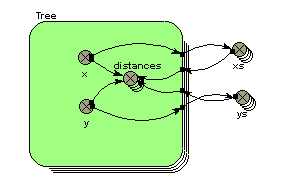Each tree has x,y co-ordinates. These are exported to two array variables, xs and ys, whose equations are simply:

xs = [x]

and

ys = [ys]

These arrays are then brought back into the submodel, and used to generate an array containing the distance for each tree to all the other trees, using the equation given in the third example above.

# Built-in functions : fmod function

fmod(X,Y)

Returns remainder after dividing X by Y

Inputs: numeric, numeric

Result: numeric

Examples:

fmod(7,3) --> 0.333 (7/2 = 2.333, i.e. the remainder is 0.333)

fmod(time(1),1) --> a result that climbs from 0 to 1 repeatedly (i.e. a sawtooth pattern) as the simulation proceeds. See comments below.

fmod((index(1)-1),5)+1 --> 1,2,3,4,5,1,2,3,4,5,1,2,3... for successive values of index(1). See comments below.

This apparently obscure function in fact has (at least) two very valuable uses.

First, it can be used to generate regular cycles, in particular annual or daily cycles. Consider the case or a model with the time unit being one year, and a time step of less than a year. You want various exogenous variables (such as temperature or rainfall) to vary in a prescribed fashion during the course of each year, with the annual pattern repeating itself from one year to the next. The following diagram is typical of the model fragment you could use for representing this:The variable time is simply set equal to current simulation time, using the function time(1). The variable season is set to rise from 0 to 1 every year. If your model used a time unit of one week, then the equation would be changed to

season = fmod(time,52)

and the value for season would then correspond to week number. The equations for rainfall and temperature are for illustration purposes only: you would need to replace them by something appropriate.

Second, the fmod function can be used to generate a regular spatial arrangement (rows and columns) for a 2D grid. Let's say that you are modelling an area of 10x10 grid squares. In Simile, you would set up a submodel, called perhaps Patch, with 25 instances. In order to give each patch location on a grid, each one needs to have a row and column attributes, with each patch having a unique combination of the numbers 1..5 for row and column. The only thing we know about each patch is that it has an index number (given by the built-in function index(1)), a value ranging between 1 and 25. The trick is to get row number to be, in sequence,

1,1,1,1,1,2,2,2,2,2,3,3,3...

and column number to be, in sequence,

1,2,3,4,5,1,2,3,4,5,1,2,3...

thus giving each of the 25 instances a unique row-and-column pair.

This is readily done using the following two equations:

row = floor((index(1)-1)/5)+1

column = fmod((index(1)-1),5)+1

See the floor function to understand why the row numbers should be in the first sequence above. For column, we divide the index number for each instance by 5, taking the remainder: the '-1' and +1' are there to ensure that we get the results in the blocks of five that we require. See a grid-based spatial model example to see this in action.

# Trigonometric functions

 Input(s) acos(X) Returns the arccos (inverse cosine) of X. Result is in radians. asin(X) Returns the arcsine of X. Result is in radians. atan(X) Returns a value in radians (range -pi/2 to pi/2), being the arctangent of X (the ratio of two sides of a right triangle). Same as arctan(X). atan2(X,Y) Returns the arctangent of X. Result is in radians. numeric,numeric cos(X) Returns the cosine of X (an angle in radians) cosh(X) Hyperbolic cosine of X. hypot(X,Y) Returns length of hypotenuse of triangle with base X and height Y numeric, numeric sin(X) Returns the sine of the argument (an angle in radians) sinh(X) Hyperbolic sine of X. tan(X) Returns the tan of the argument (an angle in radians) tanh(X) Hyperbolic tangent of X.

List handling

# Built-in functions : product function

product function

product([X])

product({X})

Result is the product of all elements of the array [X] or the list {X}

Input: numeric array or list

Result: numeric

Example:

product([2,3,4]) --> 24

# Built-in functions : place_in function

place_in function

place_in(I)

When making an array with the makearray function, place_in() returns the current position in the array. If makearray() functions are nested one inside another, the argument to the place_in() function will determine which position is returned. An argument of 1 refers to the innermost makearray().

Input: integer

Result: integer

Examples:

makearray(if place_in(1)==1 then 10 else 5, 4) --> [10, 5, 5, 5]

makearray(if place_in(1)==2 then 10 else 5, 4) --> [5, 10, 5, 5]

makearray(makearray(if place_in(2)==1 then 10 else 5, 4), 2) --> [[10, 10, 10, 10], [5, 5, 5, 5]]

makearray(makearray(if place_in(1)==1 then 10 else 5, 4), 2) --> [[10, 5, 5, 5], [10, 5, 5, 5]]

makearray(4*place_in(1),12) --> [4 8 12 16 20 24 28 32 36 40 44 48]

makearray(makearray(place_in(1)*place_in(2),12),12) -->

[[1 2 3 4 5 6 7 8 9 10 11 12],

[2 4 6 8 10 12 14 16 18 20 22 24],

[3 6 9 12 15 18 21 24 27 30 33 36],

[4 8 12 16 20 24 28 32 36 40 44 48],

[5 10 15 20 25 30 35 40 45 50 55 60],

[6 12 18 24 30 36 42 48 54 60 66 72],

[7 14 21 28 35 42 49 56 63 70 77 84],

[8 16 24 32 40 48 56 64 72 80 88 96],

[9 18 27 36 45 54 63 72 81 90 99 108],

[10 20 30 40 50 60 70 80 90 100 110 120,

[11 22 33 44 55 66 77 88 99 110 121 132],

[12 24 36 48 60 72 84 96 108 120 132 144]]

This function is only meaningful inside makearray() function. If used elsewhere, the equation parser will signal an error.

# Built-in functions : makearray function

makearray function

makearray(X,N)

Makes an array consisting of N lots of X

Input: any type, numeric

Result: array of same type

Examples:

makearray(7, 3) --> [7, 7, 7]

makearray([rand_var(0, 1), rand_var(0, 5)], 5) --> [[0.62352, 2.43459], [0.11933, 0.423529], [0.94208, 4.43623], [0.40088, 1.63023], [0.11769, 4.97782]]

This is an array constructor. The first argument can be any expression, and the second is an integer. The result is an array, each of whose elements is generated by evaluating the first argument. The size of the array is the value of the second argument, which must be a constant. If the first argument is an array, the result will be an array of arrays. See also the place_in function, which is used in complex constructions with makearray.

Use of makearray() is called explicit replication. It differs from implicit replication in that the expression being replicated is evaluated separately for each member of the generated array, including any implicit (but not explicit) intermediate results. This means that no attempt is made to combine the dimensions of the two arguments. The second argument must be a scalar integer, and the result will be an array whose outermost dimension is that value, and whose inner dimensions are the dimensions of the first argument.

makearray() could have been designed to work differently on an array first argument, replicating each element rather than the whole array. As in the case of implicit replication, the actual behaviour was chosen to be that which would be hardest to achieve by combining other functions. If you need to replicate the elements of an array, you can first split it up with the element() function then rejoin the results with makearray, e.g., makearray(makearray(element([3,6,9],place_in(2)),2),3) -> [[3,3],[6,6],[9,9]], whereas if makearray itself worked like this, it would be very hard to get its actual behaviour.

# Built-in functions : with_greatest function

with_greatest function

with_greatest([N], [X])

with_greatest({N}, {X})

Returns the value from an array [X] or the list {X} whose position in the array or list corresponds to the largest value in the array [N] or list {N}.

Inputs: numeric array or list, same dimensioned array or list with members of any type

Result: single value from second input

Example:

with_greatest([2,5,7,3], ["red", "blue", "green", "yellow"]) --> "green"

This example would require the definition of an enumerated type with the members "red", "blue", "green" and "yellow".

# Built-in functions : sum function

sum function

sum([X])

sum({X})

Result is the sum of all elements of the array [X] or the list {X}

Input: numeric array/list

Result: numeric

Example:

sum([2,3,4]) --> 9

sum([[1,2],[3,4]]) --> [4,6]

Comment:

Note the behaviour with nested arrays. A new array results, consisting of the sum of the first value of each array, the sum of the second value of each array, etc.

sum function

sum([X])

sum({X})

Result is the sum of all elements of the array [X] or the list {X}

Input: numeric array/list

Result: numeric

Example:

sum([2,3,4]) --> 9

sum([[1,2],[3,4]]) --> [4,6]

Comment:

Note the behaviour with nested arrays. A new array results, consisting of the sum of the first value of each array, the sum of the second value of each array, etc. The reason it is implemented this way is that the converse (generating an array of the sums of each sub-array in the original, i.e., [3,7] in the above example) is easier to generate explicitly if required. In the case where the 2-D array is a value coming out of nested 1-D submodels, it can be produced by putting the sum() function inside the outer submodel. If the array is only available as a 2-D value, the same effect can be produced using the element() and makearray() functions as follows:

makearray(sum(element([[1,2],[3,4]], place_in(1))),2) --> [3,7]

All other cumulative functions behave the same way regarding selection of elements from multidimensional arrays.

# Built-in functions : order function

### order function (new in v6.9)

Takes an array of numeric values and returns an array containing the indices of those values in ascending order.

Example:

order([1,9,2,10,3,8,5]) -> [1,3,5,7,6,2,4]

Note that the result of order() can be used to get the original values in ascending order by using it in the element function. e.g.,

mixed = [1,9,2,10,3,8,5]

sort = order(mixed)

element([mixed],[sort]) -> [1,2,3,5,8,9,10]

# Built-in functions: interpolate function

interpolate(X, [Xarray], [Yarray])

X is an input value.   The arrays Xarray and Yarray define a series of coordinates.   They must contain the same number of elements, and the values in Xarray must be in ascending order.   If the value of X is less than the first element of Xarray, then the result is the first element of Yarray.     If the value of X is greater than the last element of Xarray, then the result is the last element of Yarray.   Otherwise, the result is the value obtained by linear interpolation between the two points which bracket the value of X.

Examples:
interpolate(3, [2,4,7], [10,20,30])  --> 15  (linear interpolation between the points (2,10) and (4,20))
interpolate(1, [2,4,7], [10,20,30])  --> 10  (X value is less than first element of Xarray, so use first element of Yarray)
interpolate(9, [2,4,7], [10,20,30])  --> 30  (X value is greater than first element of Xarray, so use last element of Yarray)

# Built-in functions: rankings function

rankings function

Takes an array of numeric values, and returns an array with the ranks of the corresponding elements in the argument. This is 1 for the largest element, and equal to the size of the array for the smallest.

Example:

rankings([1,9,2,10,3,8,5]) -> [7,2,6,1,5,3,4]

# Built-in functions: subtotals function

subtotals function

Takes an array of numeric values, and returns an array containing the running totals from summing the elements in the original array.

Example:

subtotals([1,9,2,10,3,8,5]) -> [1,10,12,22,25,33,38]

# Built-in functions : element function

element function

element([X],I)

Picks the Ith value from the array [X]

Inputs: an array [X] of any type

integer I

Result: type

Examples:

element([10,20,30,40],3) --> 30 (since 30 is the value of the 3rd element of the array.

element([[1,2], [3,4], [5,6]],2) --> [3,4] (since the array [3,4] is the 2nd element of the input array)

element([10,20,30,40],index(1)) --> 10 for the first instance of a four-instance submodel, 20 for the second instance, etc, since index(1) has the value 1 for the first instance, 2 for the second instance, etc.

This is an essential function for use with multiple-instance submodels, in which case it is almost always used in combination with the function index(1) in the second argument. One common use is to provide each instance of a multiple-instance submodel with a unique value for some parameter or other value. The third example (above) illustrates this: that could, for example, be the expression in a compartment element inside a four-instance submodel, initialising the compartment for each of the four instances to 10, 20, 30 and 40 respectively.

The element function has rather more power than suggested above. The second argument can act as a sort of template to say how values (or sub-arrays) from the first argument are to be picked up. This is illustrated by the following example:

element([3,2,7,4,9,34,1,5], [[5,2], [1,5]]) --> [[9,2], [3,9]]

If the first argument is multidimensional and the second argument is a one-dimensional array, the elements of the second argument will be used to pick elements from the innermost arrays of the first argument from whuch to build the result, e.g.,

element([[3,5,11], [1,2,8]], [2,1,2]) --> [1,5,8].

The reason it works this way is that the converse behaviour, i.e., each element of the second argument selecting a value from the corresponding element of the first argument, is easy to get in the case where the first argument is a value from a nested submodel by putting the element() function inside the outer submodel, and can be obtained in general by building up from simple cases, e.g.,

makearray(element(element([[3,5,11], [1,2,8]],place_in(1)), element([3,1], place_in(1))), 2) --> [11,1].

makearray(element([[5,7],[1,4],[8,5]], element([3,3,2,2], place_in(1))), 4) --> [[8,5], [8,5], [1,4], [1,4]]

Use of element() on lists

Starting with Simile version 6.1, it is possible to have a list-valued expression as the first argument of element(), in which case the result is a sublist of that list, i.e., a list containing some, all or none of the members of the original list in the same order. The second argument can be a single value, in which case the resulting list has one element if the list includes a value with that index, and none otherwise. So applying sum() to it gives either a value from the original list or zero.

If the second argument is an array or list, the result is a sublist of the first argument containing all the values whose indices appear as values in the second argument. There are a few points to note about all these uses:

• It is not computationally efficient. The values from the list are found by searching through it sequentially rather than by lookup as can be done on arrays.
• If the second argument is an array or list, its values must be in ascending order, or in the order in which they appear in the definition if they are of an enumerated type. If a value occurs more than once in the second argument, the value with that index will still only appear once in the result. This is because rather than searching the list from the start for each value, the generated code merely starts from where the last one was found, since the indices in the original list should always be in ascending order. (This does not apply when selecting a sublist from a list of neighbour values in a special-purpose submodel; the direction identifiers can be in any order, and if one occurs more than once, its value from the original list will also be repeated).
• The resulting list cannot itself be used as the first argument of element(), or arithmetically combined with other list-valued expressions.

element() with multiple indices

Starting with Simile version 6.1, if you have a 2-D (or higher) array, you can look up a single member by using element() with 3 (or more) arguments, e.g.,

element([[arr]], x, y)

Formerly you would have had to do this by nesting element() calls, but the new format is neater and allows the indices to be matching arrays or lists themselves to get multiple values.

Examples:

```
element([[6,1,8],[7,5,3],[2,9,4]], 2, 2) --> 5```
`element([[6,1,8],[7,5,3],[2,9,4]], [2,1,3], [3,1,2]) --> [3,6,9]`
```
```

# Built-in functions : any function

any function

any(([X])

any({X})

Result is true if any of the elements of the array [X] or the list {X} are true.

Input: boolean array or list

Result: boolean

Examples:

any([false,false,false,true,false]) --> true

any([false,false,false]) --> false

# Built-in functions : all function

all function

all([X])

all({X})

Result is true if all the elements of the array [X] or the list {X} are true

Input: boolean array/list

Result: boolean

Examples:

all([true,true,true,false]) --> false

all([true,true,true,true]) --> true

# Built-in functions : least function

least function

least([X])

least({X})

Returns the smallest value from an array [X] or the list {X}

Inputs: numeric array/list

Result: numeric

Example:

least([2,5,7,3]) --> 2

# Built-in functions : greatest function

greatest function

greatest([X])

greatest({X})

Returns the largest value from an array [X] or the list {X}

Inputs: numeric array or list

Result: numeric

Example:

greatest([2,5,7,3]) --> 7

# Built-in functions : with_least function

with_least function

with_least([N], [X])

with_least({N}, {X})

Returns the value from an array [X] or the list {X} whose position in the array or list corresponds to the smallest value in the array [N] or list {N}.

Inputs: numeric array or list, same dimensioned array or list with members of any type

Result: single value from second input

Example:

with_least([2,5,7,3], ["red", "blue", "green", "yellow"]) --> "red"

This example would require the definition of an enumerated type with the members "red", "blue", "green" and "yellow".

Model properties

# Built-in functions : after function

after function

after(T, M)

Use only as whole equation of derived event. Instead of firing immediately when triggered, event is delayed by value of 1st argument, then fires with magnitude the 2nd argument had when triggered.

Inputs: Real, Any data type

Result: Same type as 2nd arg

# Built-in functions : last function

last function

last(X)

Recalls value of X, another element, from previous time step. X must influence this element in order to be used in the equation. See prev, for a function that returns a previous value from this element itself.

This function has been replaced with the const_delay( ) and var_delay( ) functions, which are more general in allowing the value of a variable to be returned from an arbitrary number of time steps before.

Input: numeric

Result: numeric

# Built-in functions : as_number function

as_number function

as_number(N)

Converts integral types to integer

Input: Boolean, enumerated type or integer (for flexibility)

Result: Value of argument as integer

# Built-in functions : prev function

prev function

prev(N)

Returns the value of this element itself, N time steps ago. See last, for a function that returns a previous value of any element other than this one itself.

Input: numeric

Result: numeric

Example:

Consider a variable that flips from state 1 to state 2 when some triggering condition is satisfied (the Boolean variable, trigger, is true, for example) then stays in state 2. The equation for the variable could make use of prev, as follows:

if time()==0 then 1 elseif trigger then 2 else prev(1)

# Built-in functions : parent function

parent function

parent(1)

Returns the id (instance number) of the individual whose reproduction gave rise to this one, or 0 if the individual being considered was created at the start of the simulation or by immigration.

Input: numeric (but a dummy value: use the value 1).

Result: integer (in fact, a negative integer number, being the instance number of a member of a population submodel. These are all numbered from -1 downwards.)

Comment:

This function is vital for the simulation of any form of biological inheritance from one generation to the next. You have to know who the parent is before you can allow the newly-created individual to inherit (possibly with modification) one or more of the characteristics of the parent.

# Built-in functions : time function

time function

time()

Returns the current simulation time.

Input: none

Result: numeric (units = day)

Comment:

Any model which has exogenous variables (variables that change as a function of time, independently of the behaviour of the model, such as air temperature or rainfall) needs some way of knowing what the current clock time is: i.e. how far the simulation has proceeded. This function provides that information.

This function is not strictly-speaking necessary: you could get exactly the same behaviour by having a single compartment, initialised to zero, with a single flow in, with a constant value of 1. So, after 1 time unit the value of the compartment would be 1, after 12.5 it would be 12.5, and so on. However, the function is provided to avoid cluttering up the model with an extra compartment and flow.

# Built-in functions : delay1, delay3, delayn functions

delay1, delay3, delayn functions

delay1(input, duration [, initial])

delay3(input, duration [, initial])

delayn(input, duration, n [, initial])

Arguments:

input: the value to be delayed

duration: time by which to delay the input value

n (delayn only): order of the material delay (delayn only)

initial (optional): the value of the result when the function first applies

Result:

The delayed value of input.

The delay1, delay3 ​and delayn function calculates a first, third or nth-order material delay of input, using an exponential delay time of delay duration, and an optional initial value initial for the delay. delay3 does this by setting up a cascade of three first-order material delays, each with a delay duration of delay duration/3. Other versions of the function behave analogously. delay3 returns the value of the final delay in the cascade. If you do not specify an initial value initial, all functions assume the value to be the initial value of input.

The delay3 function will return the value of delay 3 in the structure and equations shown in the following figure:Compartment   comp1 :
Initial value = start_fill (real)
Compartment   comp2 :
Initial value = start_fill (real)
Compartment   comp3 :
Initial value = start_fill (real)
Flow   delay 1 :
delay 1 =         comp1*3/duration (1/day)
Flow   delay 2 :
delay 2 =         comp2*3/duration (1/day)
Flow   delay 3 :
delay 3 =         comp3*3/duration (1/day)
Flow   inflow :
inflow =         input (1/day)
Variable   start fill :

start fill =         initial*duration/3 (real)

Example:

Delay 3 = delay3(input, 5) where input = 5 + step(10,3) produces the pattern shown below: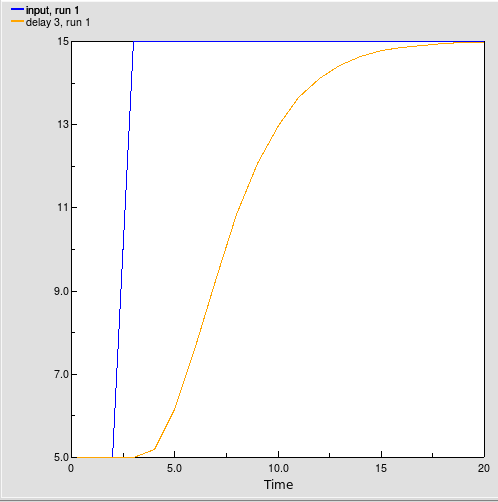# Built-in functions : first function

first function

first(T)

Takes an argument T that is a member of an enumerated type, and returns "true" if it is the first member of its type, and "false" otherwise.

Input: enumerated type member, or array of enumerated type members

Result: boolean, or array of boolean values

Examples:

If enumerated type "fruit" is defined as "apple", "grape", "banana":

first("apple") --> "true"

first(["banana", "apple", "banana"]) --> ["false", "true", "false"]

# Built-in functions : following function

following function

following(T)

Takes an argument T that is a member of an enumerated type, and returns the next member of the enumerated type.

Input: enumerated type member, or array of enumerated type members

Result: enumerated type member, or array of enumerated type members

Examples:

If enumerated type "fruit" is defined as "apple", "grape", "banana":

following("apple") --> "grape"

following(["grape", "apple", "grape"]) --> ["banana", "grape", "banana"]

# Built-in functions : forcst function

forcst(<input>,<time>,<horizon>[,<initial>])

New in v6.6

The forcst function performs simple trend extrapolation. Here's how it works. First, forcst calculates the trend in input, based upon the value of input, the first order exponential average of input, and the averaging time. (Think of the averaging time as the time over which you wish to calculate a trend.) Then forcst extrapolates the trend into the future - you specify the distance into the future by providing a value for horizon. If you do not specify initial, forcst substitutes 0 for the initial value of the trend in input.

The forcst function is equivalent to the structural diagram and equations shown in this figure:Compartment   Average input :

Initial value = input-(averaging*initial) (real)
Flow   change in average :
change in average =         (input-Average_input)/averaging (1/day)
Variable   averaging :
averaging =         Variable parameter (day)
Variable   forecast :
forecast =         input*(1+trend*horizon) (real)
Variable   horizon :
horizon =         Variable parameter (day)
Variable   initial :
initial =         Variable parameter (real)
Variable   input :
input =         Variable parameter (real)
Variable   trend :
trend =         (input-Average_input)/(Average_input*averaging) (1/day)

Example:

Sales_Forecast = FORCST(Sales,10,15,0) produces a forecast of sales 15 time units into the future. The forecast is based on current sales, and the trend in sales over the last 10 time units. The initial growth trend in sales is set to 0.

# Built-in functions : in_preceding function

in_preceding function -- new in Simile v5.7

Usage: in_preceding(expression of any type) returns that type

Definition: Used in a multi-instance submodel, returns the value of the argument expression  as it would be in the preceding instance of that submodel, or 0 or "false" in the first instance. The argument can include the function prev(0) to refer to the value in the previous submodel instance of the component in whose equation the in_preceding() function appears.

Note that a model that contains a circular set of influences can build and run properly if the input parameter associated with one of the influences is only used in the argument of an in_preceding() function. This is because since the value of the argument is calculated for one submodel instance and then used in the next, there is no actual circular dependency.

Example 1:

A 5-instance submodel contains a variable with the equation

index(1)+in_preceding(prev(0)).

The values will be:

1 3 6 10 15

Example 2:

An 8-instance submodel contains two variables, "received" and "forwarded". These are connected to one another by influences in each direction. The equation for "forwarded" is received/2. The equation for "received" is

if index(1)==1 then 200 else in_preceding(forwarded)

The values of "received" for the 8 instances will be:

200 100 50 25 12.5 6.25 3.125 1.5625
In: Contents >> Working with equations >> Functions >> Built-in functions

# Built-in functions : ramp function

ramp(time,slope)

Generates a ramp of slope slope, starting at time time and zero before that time.

Result: the ramp function.

Arguments: time at which to start ramping, slope (positive or negative) of ramp

Example:

the function ramp(20,-7) will have a return value of 0 at time 20 and -70 at time 30

# Built-in functions : smth1, smth3, smthn functions

smth1, smth3, smthn functions

smth1(input, averaging [, initial])

smth3(input, averaging [, initial])

​smthn(input, averaging, n [, initial])

Arguments:

input: the value to be smoothed

averaging: time over which to smooth the input value

n (smthn only): order of the material smoothing

initial (optional): the value of the result when the function first applies

Result:

The smoothed value of input.

The smth1, smth3 and smthn functions perform a first-, third- and nth-order respectively exponential smooth of input, using an exponential averaging time of averaging, and an optional initial value initial for the smooth. smth3 does this by setting up a cascade of three first-order exponential smooths, each with an averaging time of averaging/3. The other functions behave analogously. They return the value of the final smooth in the cascade. If you do not specify an initial value initial, they assume the value to be the initial value of input.

The smth3 function will return the value of comp3 in the structure and equations shown below.Compartment   comp1 :
Initial value = initial (real)

Compartment   comp2 :
Initial value = initial (real)

Compartment   comp3 :
Initial value = initial (real)

Flow   flow1 :
flow1 =         (input-comp1)*3/averaging (1/day)

Flow   flow2 :
flow2 =         (comp1-comp2)*3/averaging (1/day)

Flow   flow3 :
flow3 =         (comp2-comp3)*3/averaging (1/day)

Examples:

Smooth_of_Step = smth3(Step_Input,5)

where

Step_Input = 5 + step(10,3) produces the pattern shown below.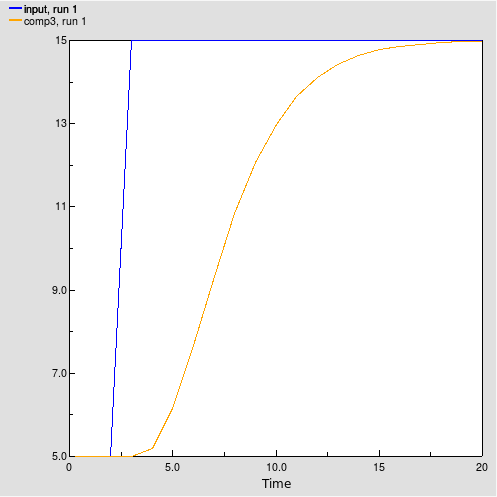# Built-in functions : step function

step(height,time)

creates a step function. Output is 0 up until time, and equal to step thereafter.

Result: the step function.

Arguments: height of step, time at which to step.

Example:

step(30, 20) has output 0 at time 19, and 30 at time 20 and after

# Built-in functions : stop function

stop function

stop(n)

Input: is a number (real or integer) of your choice

Result: None (see text)

When executed, this function halts execution of the model, and produces the following error message:

Simile ran into a problem trying to run this model.

While it was trying to calculate the value of variable

var (node x) during execution of the model at

time t, there was a user-defined interruption: n.

It is useful to define error conditions where you (the model designer) know that the model should not be used or is not applicable for some reason. Trivially, it can be used to guard against mathematical errors, for example:

if (time()-50) != 0 then 1/(time()-50) else stop(5)

This form has some merit when running in C++, but generally, to track down mathematical errors, it is easier to debug in Tcl. If execution in Tcl would take too long, then this is a useful alternative. Its primary use however, is to enable you to catch out-of-range conditions in the specific circumstances of your model.

The use of an error code in the user-defined interruption (e.g. stop(13) ) enables you to see which stop( ) function caused the model to stop running, if there is more than one in your model.

Result is undefined, because simulation stops at the point at which the function is called, but has integer type (this is important because if it is called in a conditional, the other branch of the conditional must also have a numerical type).

Examples:

if population>50 then stop(1) else 0

if (time()-50) != 0 then 1/(time()-50) else stop(5)

# Built-in functions : trend function

trend(<input>,<time>[,<initial>])

New in v6.6

The trend function calculates the trend in input, based upon the value of input, the first order exponential average of input, and the exponential averaging time averaging time. trend is expressed as the fractional change in input per unit time. If you do not specify initial, trend substitutes the value 0 for the initial value of the trend.

The trend function is equivalent to the structural diagram and equations shown in this figure:Compartment   Average input :

Initial value = input-(averaging*initial) (real)
Flow   change in average :
change in average =         (input-Average_input)/averaging (1/day)
Variable   averaging :
averaging =         Variable parameter (day)
Variable   initial :
initial =         Variable parameter (real)
Variable   input :
input =         Variable parameter (real)
Variable   trend :
trend =         (input-Average_input)/(Average_input*averaging) (1/day)

Example:

Yearly_Change_in_GNP = TREND(GNP,1,.04)

This equation calculates the annual change in the input GNP. It starts with an initial value of .04 (4% per year).

# Built-in functions : var_delay function

var_delay function

var_delay(var,n)

Input: a variable name and a numerical value (real or integer) of time units

Result: the value (any type) of the named variable, n time units ago

This function returns some previous value of another variable, an arbitrary period of time before. The period of time is defined in time units (not steps). The number need not be an integer, but whatever the actual time step, delay is always rounded to the nearest multiple of 0.1 of a time unit. The variable whose previous value is required is specified by name. The variables must be linked with an influence arrow.

This is a general replacement for the last( ) function, which returns the value of the named variable from the previous time step only. The delay must be between 0 and 100 and is rounded to the nearest 0.1. The delay can vary; if the delay is constant, the function const_delay() will do the job more efficiently.

Examples:

runoff=var_delay(rain,soak_time)

# Built-in functions: at_init function

at_init function

at_init(X)

Returns the value the argument had when first used, i.e., on model reset or when the submodel instance containing this equation was created.

at_init(X) creates an implicit intermediate result, which has the same dimensions as its argument. So if this result is implicitly replicated elsewhere in the equation, the same value will be used each time. See makearray for behaviour in explicit replication.

Input: Any data type

Result: Same type as input

# Built-in functions: at_posn function

at_posn function

at_posn(C)

at_posn(C,Row,Col)

Must form the whole equation of a component. Sets the component's value to the value of a component in an instance of a 2-D submodel representing a grid. C is the caption of the component in the grid submodel, and Row and Col if present are the outer and inner indices of the source instance (i.e., grid square) from which to get the value. If Row and Col are not present, the grid is mapped onto the diagram of the submodel containing the target component and the source instance selected by the component's position in the submodel diagram.

Input: Any data type plus optionally two integers

Result: Same type as input

# Built-in functions: const_delay function

const_delay function

const_delay(var,n)

Input: a variable name and a numerical constant (real or integer) of time units

Result: the value (any type) of the named variable, n time units ago

This function returns some previous value of another variable, a arbitrary period of time before. The period of time is defined in time units (not steps). The number need not be an integer, but whatever the actual time step, delay is always rounded to the nearest multiple of 0.1 of a time unit. The variable whose previous value is required is specified by name. The variables must be linked with an influence arrow.

This is a general replacement for the last( ) function, which returns the value of the named variable from the previous time step only. The delay must be a numeric constant; for variable delay see var_delay().

Examples:

runoff=const_delay(rain,10)

# Built-in functions: dies_of function

dies_of function

dies_of(X)

Returns true if argument is the loss channel that will cause the individual to disappear at the end of the current time step.

Input: value from a loss channel in the local submodel

Result: boolean

# Built-in functions: in_progenitor function

in_progenitor function -- new in Simile v5.8

Usage: in_progenitor(expression of any type) returns that type

Definition: Used in a population submodel, returns the value of the argument expression  as it would be in the instance of that submodel containing the reproduction channel responsible for the instance being evaluated, or 0 or "false" in an instance that arrived via a channel other than reproduction. The argument can include the function prev(0) to refer to the value in the progenitor submodel instance of the component in whose equation the in_progenitor() function appears.

Note that a model that contains a circular set of influences can build and run properly if the input parameter associated with one of the influences is only used in the argument of an in_progenitor() function. This is because since the value of the argument is calculated for the progenitor instance and then used in the offspring instance, and the progenitor always comes before the offspring in evaluation order, there is no actual circular dependency.

Important: If the progenitor instance has been removed (see Extermination) then using this function will return meaningless values, and may cause model execution to be aborted due to memory access violations. To avoid this problem, make the in_progenitor function itself the argument of an at_init() function, e.g., at_init(in_progenitor(index(1))). If this is done, the inner argument will be evaluated for the progenitor instance when the offspring instance is created -- at which time the progenitor definitiely exists -- and then retained within the offspring instance's data structure. The only reason for not doing this would be if changes in the value in the progenitor continue to affect the offspring, and offspring never outlive their progenitors, e.g., in an L-systems model of tree branching.

# Built-in functions: iterations function

iterations function

iterations(X)

Returns number of iterations that have been done up to this point in an alarm submodel. Argument is the boolean balue from the alarm symbol.

Input: value from an alarm symbol in the local submodel

Result: integer

# Built-in functions: preceding function

preceding function

preceding(T)

Takes an argument T that is a member of an enumerated type, and returns the previous member of the enumerated type.

Input: enumerated type member, or array of enumerated type members

Result: enumerated type member, or array of enumerated type members

Examples:

If enumerated type "fruit" is defined as "apple", "grape", "banana":

preceding("grape") --> "apple"

preceding(["banana", "grape", "banana"]) --> ["grape", "apple", "grape"]

# Built-in functions: pulse function

New in v6.6. Note this function is provided purely for compatibility with continuous-only modelling tools, and new models should use squirts instead.

pulse function:

pulse(magnitude, first_time [, interval])

Generate a pulse with a duration of a single time step and a given cumulative value.

Result: the pulse waveform

Arguments:

magnitude: the cumulative value of the pulse. This will be the level change of a compartment due to a flow with this value coming into it.

first_time: The first, last or only time at which the pulse will occur

interval (optional) : If this is positive, the pulse will occur regularly with this interval after the initial time

If it is negative, the pulse will occur with this interval up until the initial time and not after

If zero or not present, the pulse will occur only once at the initial time

Example: pulse(20, 12, 5) generates a pulse value of 20/DT at time 12, 17, 22, etc.

# Built-in functions: trigger_magnitude function

trigger_magnitude function

trigger_magnitude()

Returns a value representing the magnitude of the triggering event. Can only be used in the equations of derived events, squirts and rule-based state variables. Events influencing these components are not listed as parameters so this function is used to get their values. If the trigger is a simple event, this function gives the value of that event's equation. If it is a time series, it is the current value from the series. If it is a limit event then it is 'true' if there is only one limit, and -1/1 for lower/upper limit if both are given.

Inputs: none

Result: same units and dimensions as triggering events

# Built-in functions : sofar function

sofar function

sofar([X])

sofar({X})

Result is…

Input: numeric array/list

Result: numeric

Example:

Comment:

# Built-in functions : size function

size function

size(S)

size(S,I)

The first form takes the name of a fixed-membership submodel and returns the number of instances that it has. The second form takes the name of a fixed-membership submodel and returns the size of the Ith level of nesting of this submodel.

Input: submodel name

Result: integer

# Built-in functions : dt function

dt function

dt(I)

Returns the duration of the level I time step.

Input: numeric. This is the time step level.

Result: numeric

Examples:

dt(1) --> 0.1 (for a model whose top-level time step was set to 0.1 in the Run Control panel)

dt(2) --> 0.001 (for a model whose 2nd-level time step was set to 0.001 in the Run Control panel)

If you are starting off with Simile, it is unlikely you will need to understand the concept of "time step index". You will probably just be making models with a single time step, hence one level, so don't worry about anything except the use of dt(1).

The main use of the dt function is to engineer the addition or removal of a specified amount of a substance into or out of a compartment. The only handle we have for causing changes to the amount in a compartment are flows, and flows are expressed as a rate per unit of time (whatever time unit is used for the model, e.g. year). This creates a problem if we want to add or remove a specified amount of substance at some instant in time. For example, consider a model with a time unit of year, a time step of 0.1, and with a compartment X from which we want to remove 5 units at the instant that some condition, which only lasts for 1 time step (0.1 years), is met. If we simply had a flow out that was zero when the condition was not met, and was 5 when the condition was met, then for one time step the flow would be 5 (units per year): hence, only 0.5 units would be removed in the 1/10th of a year, not the 5 we intended. What we need to do is to artificially inflate the flow rate by a factor of 10 (in this case, with a time step of 0.1) for this one time step. We do this by dividing the flow rate (in our flow equation) by 0.1 (in this case), or by dt(1) in general. The flow rate then appears to be 50 units per year for that one time step, giving a loss of 5 units in the one time step. Bingo!

The actual flow expression for the case considered above would be:

flowout = 5/dt(1)

Special case

Using the argument zero in the function, i.e. dt(0), is equivalent to saying dt(n), where n is the time step index of the submodel in which the function is used. This is useful, because when a submodel time step index is changed, it is then not necessary to edit the dt() functions within it to preserve the meaning.

# Built-in functions : count function

count function

count([X])

count({X})

Number of values in the array [X] or the list {X}

Input: array or list of values (numeric or boolean)

Result: integer

Examples:

count([4,5,6]) --> 3

# Built-in functions : channel_is function

channel function

channel_is(X)

X is an immigration, reproduction or creation channel. Returns true if this individual appeared through that channel.

Input: numeric

Result: Boolean

Examples:

channel_is(cr1) --> true, for each instance of the population that was initially created through channel cr1.

This function can only be used inside population submodels. Its argument is the name of a channel (i.e. a population control symbol, one of creation, immigration or reproduction). Note that the value of the channel itself is not used, just the name. The result can be used in calculations inside the population submodel. For example, the expression

land_owned = if channel_is(im1) then 0 elseif channel_is(cr1) then 10

would allocate 10 acres of land to each member of the original population, but none to immigrants.

# Built-in functions : init_time function

init_time function

init_time(1)

Returns the time at which this model component first came into existence. This really only has any use for:

• population submodels, so that the model knows when a new member of the population was created; and
• conditional submodels, so that the model knows when the submodel, or one instance of it, came into existence because the condition controlling it became true.

Input: numeric. In fact, the argument is not used, so simply insert the number 1. The only reason for having the brackets and an argument enclosed between them is that this is the only way that Simile can recognise that this is a function.

Result: numeric

Example:

Let's assume you have a population submodel, and some property of each individual is related to its age (e.g. its growth rate, or its probability of dying). Simply create a variable called age (inside the submodel), and insert the following equation:

age = time(1) - init_time(1)

The result is the difference between the current simulation time (given by the function time(1), and the time when the instance was created, given by init_time(1).

# Built-in functions : index function

index function

index(I)

Returns the index (instance number) of a member of a fixed membership or population submodel, for the level of submodel nesting specified by the argument.

Input: numeric

Result: numeric

Comment:

The index function is frequently used in conjunction with the element function when working with multiple-instance submodels: both fixed-membership and population submodels.

The argument specifies which index is to be returned. You can see summary information about the meanings of the different indices in the listbox headed Indices: in the equation dialogue. The argument is an integer between 1 and the maximum number of indices available. index(1) corresponds to the 'innermost' index, i.e., if you have one multiple-instance model inside another, the result of index(1) will be the index of the inner submodel instance, and the result of index(2) will be that of the outer submodel instance. Similarly, if a submodel has two dimensions, then index(1) and index(2) will be valid in that submodel, giving an instance's position along the inner and outer dimension respectively.

Relation submodels do not usually have indices of their own, but you can get the indices of their base submodel instances using the index() function. If one of the roles in a relation has been specified to 'allow base instance lookup', then the base submodel for this role will be 'innermost' and and the index of the instance in this role will be the result of calling 'index(1)' in the relation submodel.

For fixed-membership submodels, the function returns an integer value between 1 and n, where n is the number of instances for the submodel. For variable-membership submodels, it returns an integer between 1 and n, where n is the maximum possible index. For a population submodel this would be the total number of instances of the population that have ever existed during this simulation run. For a conditional submodel the maximum will be the size given in the submodel dimensions. For either of these, an instance with a particular index number may or may not exist.

# Built-in functions : Statistics

Statistical functions produce variates from distributions, and generally produce different values at each point in the model where they are called.

There are up to three forms of each statistical function:

• The form with a _const suffix. This will produce a new value when the simulation is initialized or reset, or when a submodel instance containing it is created. This value stays the same until the end of the run, or until the submodel instance containing it ceases to exist.
• The form with a _var suffix (or no suffix). This will produce a new value on each time step for each instance where it occurs. The sequence of values for all such functions can be initialized using the Initialize pseudo-random tool. If there is no _const form of a function, the _const behaviour can be produced by wrapping this form in the at_init() function.
• The form with an extra argument. The last argument (an integer) serves as a seed for the values produced by that particular occurrence of the function. These will be the same each run, and independent of any other statistical functions in the model. For instance if it is used in a conditional submodel, it will start producing the same sequence of results each time an instance of the submodel comes into existence (assuming the seed value is the same).

If a statistical function only has one form, it behaves like the 'var' form.

# Built-in functions : rand_const function

rand_const function

rand_const(X,Y)

Returns a random number between X and Y at the start of the simulation or when the submodel instance is created. The random-number generator is not called again, and so the value stays the same until the simulation is reset.

Input: numeric, numeric

Result: numeric

Comment:

The main use of this function is to assign values to a set of instances of a multiple-instance submodel (fixed-membership or population). For example, to randomly assign initial sizes to a set of trees in a multiple-instance tree submodel, we could use the equation:

size = rand_const(12,20)

or to assign random locations to the trees, we could use the equations:

x = rand_const(0,50)

y = rand_const(0,100)

which would randomly place the trees in the left-hand half of a one-hectare plot, assuming that the values are in metres.

The use of rand_const() is deprecated because it cannot be made to behave in the same way as rand_var when implicitly replicating over an array. It is implemented by internal conversion to at_init(rand_var(x,y)) and this form should be used in full to make the replicatio behaviour clear.

Historical note: You may come across some models that use a rand(X,Y) function. This behaves like rand_const if Simile deduces that the model element will only be called at initialisation time, and like rand_var if the equation contains some variable that changes over time. The use of this function is now also deprecated because the semantics of the two uses are so very different. Also, there are some situations when you need to be able to over-ride this behind-the-scenes decision about how the function should behave.

# Built-in functions : rand_var function

rand_var function

rand_var(X,Y)

Returns a random number between X and Y, with a new value every time step.

Input: numeric, numeric

Result: numeric

Comment:

This function is used for doing stochastic modelling and Monte-Carlo simulations, i.e. one or more processes in the model (like giving birth or dying) have a random element to them.

rand_var gives a new result for every call, and if it is used in an expression that is replicated to make an array, each element's random value will be different.

rand_var uses the pseudo-random sequence generator built into the c++ compiler which Simile is using to create executable models. The sequence is initialized with a value generated from the process ID and clock time when Simile starts up, so no two runs will produce the same results. However, if it is required that a model has exactly the same behaviour each time it runs, despite including calls to rand_var, this can be achieved by means of a tool that sets the seed to a given value; see Initializing pseudo-random sequence.

Historical note: You may come across some models that use a rand(X,Y) function. This behaves like rand_const if Simile deduces that the model element will only be called at initialisation time, and like rand_var if the equation contains some variable that changes over time. The use of this function is now deprecated because the semantics of the two uses are so very different. Also, there are some situations when you need to be able to over-ride this behind-the-scenes decision about how the function should behave.

# Built-in functions : binome function

binome function

binome(prob, n)

Input: Real numerical value, integer value

Result: A value from the binomial distribution with the given probability and number of trials. A new random deviate is generated each time step.

The binomial distribution describes the probability of a given number of positive outcomes occurring when a number n of trials are carried out, each with a certain probability p of a positive outcome.

This function is implemented using a pseudo-random sequence generator; notes regarding its behaviour can be found in the documentation for the rand_var function.

Examples:

# Built-in functions : colin function

colin function

colin([Array])

Returns a deviate from a distribution whose relative probabilities are given by the values in the argument array. A new deviate is generated each time step.

Inputs: array of probabilities (real).

Outputs: index to value in array (int).

This can be used to make a deviate from an explicit set of probabilities where the pattern does not match any other built-in statistical function.

This function is implemented using a pseudo-random sequence generator; notes regarding its behaviour can be found in the documentation for the rand_var function.

Example:

colin([1,1,1,10,1]) --> 4 (usually), 1,2,3 or 5 (occasionally).

# Built-in functions : exprnd function

exprnd function

exprnd(mean [, seed])

Returns: value sampled from an exponential distribution (numerical)

Arguments: mean of distribution (numerical), seed for random sequence (integer, only required if a reproducible series of values is needed)

Example: A Geiger counter pointed at a radioactive source will emit a series of clicks at random times. The durations of the intervals between the clicks are distributed exponentially.

# Built-in functions : gaussian_var function

gaussian_var function

gaussian_var(mean, sd)

Input: Two real numerical values

Result: A random sample from a Gaussian (normal) distribution, with the supplied mean and standard deviation. A new random sample is generated each time step.

This function is implemented using a pseudo-random sequence generator; notes regarding its behaviour can be found in the documentation for the rand_var function.

Examples:

daily_rainfall = gaussian_var(annual_rainfall/365, 1.0)

# Built-in functions : with_colin function

with_colin function

with_colin({ProbList},{ValList})

Takes two lists with equal size, and returns an element from the second argument, picked at random with the probability of each element proportional to the value of the corresponding element in the first argument. A new return value is generated each time step.

Inputs: list of probabilities (real), list of corresponding values (any).

Outputs: element picked from second list (any).

This can be used to make a deviate from an explicit set of probabilities where the pattern does not match any other built-in statistical function.

Note that it only works on lists; if you want to do something similar with fixed-size arrays, you can combine the element and colin functions to achieve the same effect as follows: element([ValList], colin([ProbList]))

This function is implemented using a pseudo-random sequence generator; notes regarding its behaviour can be found in the documentation for the rand_var function.

Example:

with_colin({1,1,1,10,1}, {"apples", "pears", "oranges", "grapes", "bananas"}) --> "grapes" (usually), "apples", "pears", "oranges" or "bananas" (occasionally)

# Built-in functions: hypergeom function

hypergeom function

hypergeom(Pop, Mark, Sample)

Returns a deviate from a hypergeometric distribution for a given population, number of marks, and size of sample.

Inputs: Population size (int), number of marked individuals (int), size of sample from population (int)

Outputs: deviate of number of marked individuals from sample

The hypergeometric distribution tells us the range of probabilities of getting a number of "marked" individuals when taking a sample of a certain size from a population, a given number of which are "marked".

This function is implemented using a pseudo-random sequence generator; notes regarding its behaviour can be found in the documentation for the rand_var function.

Example: A research process involves ringing a certain number of seabirds from a population and releasing them, then at a later date recapturing a different number of the birds and checking how many ringed individuals are retreived. If a random group of individuals are captured each time, the probability of getting n rings back is equal to the probability of getting the result n from the equation:

rings_retrieved = hypergeom(Seabird_population, Birds_ringed, Birds_caught)

# Buit-in functions : poidev function

poidev function

poidev(mean)

Input: Real numerical values

Result: A value from the Poisson distribution with the given mean. A new random deviate is generated each time step.

The poisson distribution describes the probability of a given number of positive outcomes occurring in the limiting case of the binomial distribution, i.e., with very many trials each with a very small chance of a positive outcome.

This function is implemented using a pseudo-random sequence generator; notes regarding its behaviour can be found in the documentation for the rand_var function.

Example: A hospital serves a large community in which a certain percentage of individuals are thought to be carriers of the hospital superbug MRSA. If we admit a small number of individuals to hospital, we would expect the probability of getting a certain number of MRSA carriers in that group to be equal to the probability of getting that number as the result of this equation:

# User-defined functions

There are three different mechanisms for users to supply functions for use in expressions:

1. user-defined macros, which provide a short-hand for long or complex expressions that would otherwise have to be used repeatedly in expressions.
2. user-defined external procedures, written in a programming language such as Tcl or C++.
3. model-fragment function definitions (introduced in Simile v6), in which the behaviour of a function is implemented by a separate Simile model.

User-supplied function declarations and definitions should be put in files in the Functions directory in Simile's local data tree. This tree is created automatically when you first run Simile. Its location is:

• On Windows: "My Simile Files", under "Documents" (Windows 7 or Vista) or "My Documents" (earlier Windows versions)
• On Linux: .simile, in the user's home directory
• On MacOS: Simile, in the user's home directory

Declarations and definitions in the Functions directory in Simile's installation tree (e.g., "Program files/Simile54") are for functions treated as "Built-In", i.e., expected to be available in any Simile system. It is worth looking at these for guidance in writing your own functions, as the formats are the same.

# Macro definitions

Macro definitions provide a shorthand for long, complex expressions that would otherwise have to be used in equations, possibly in several different elements. The definitions are stored in one or more external files, which are read each time Simile starts. Users may edit the files to include user-defined macros.

Each new macro is defined in a new line in any file with the extension .pl in the Functions directory. There are two places where this directory can be; one is within the Simile program files tree (for built-in functions), and the other is under Simile's local directory (for functions to be treated as user-defined). Any functions added by modellers should be placed in the latter location;  this has the effect that when a model requiring the functions is saved, they are marked as user-defined, and if the model is subsequently opened on a system where the function definitions are not present, a warning is displayed saying which user-defined functions are missing.

The format of the macro definition line is:

f(X1, X2 ... Xn) --> F(X1, X2 ... Xn).

where:

• f is the name of the user-defined function. This has the format of a Prolog atom or variable, so it must start with a letter, and unlike the built-in function names it is case-sensitive.
• X1, X2 … Xn are a series of one or more variable identifiers. These also have the format of Prolog atoms or variables, so they must start with letters and are case sensitive. These formal arguments to the function are replaced when the function is used, by the actual argument values. If the function requires no arguments, you must place a pair of single quotes between the empty parentheses.
• F(X1, X2 … Xn) represents any expression that could be used in an element's equation. The variable identifiers can be used as quantities anywhere in this expression. This is the macro itself. As with any expression in the equation language, it may extend over more than one line.
• The symbol '-->' means 'maps onto'. It, and the final period, are part of Prolog syntax.
• Next line shows how to write 0-ary (no arguments) macro definitions (leave parentheses out) and macro definitions calling 0-ary functions or macros (put empty atom in parentheses).
init_time --> at_init(time('')).
Note the argument in the call is two single quotes, not a double quote.

The function, as it appears on the left side of the arrow, can then be used in any Simile equation, with any sub-expression taking the place of each of the variable identifiers. The result returned by the function will be the same as that which would have been returned by the expression on the right hand side, if the same sub-expressions had been substituted for the variable identifiers.

The .pl files distributed with Simile (and installed in the Functions directory in Simile's program files) contain a number of examples of function definitions. These include the following:

• subtotals(Arr): Takes an array and returns another array of the same size containing the totals of all the values up to that point in the original array. e.g., subtotals([1,2,4,3]) = [1,3,7,10].
• rankings(Arr) Takes an array and returns an array of integers of the same size each representing the position in the sequence of largest to smallest (largest = 1) of the corresponding value in the original array. e.g., rankings([8.2, -5.1, 2.5]) = [1,3,2].
• with_greatest(Arr1, Arr2) Takes two arrays and returns the element of the second in the position corresponding to the largest-valued element of the first
• colin(Arr) Takes an array and on each time step returns an integer, with the probability of each value being proportional to the value at that position in the original array.

Comment lines, starting with a %, can be included in this file, and standard multi-line comments bounded by /*...*/ can be used.

If there is a syntax error in a user function definition, this will cause a warning to be produced when Simile is started. The other definitions will still be usable.

# External procedural functions

To include user-defined external procedures, there are three files:

1. A file under the Functions directory with the .pl extension. This file can also contain macro definitions. Procedurally defined functions that are treated as being built-in to Simile go in Simile's installation tree, while those that are treated as user-defined go in the local information tree. The declarations take one of two possible forms:

function(Name, ResultType, ArgTypes).
This form is used for ordinary, deterministic functions.
sample(Name, ResultType, ArgTypes).
This form is used for functions which give a new value each time they are called, even if the arguments do not change. This is used for stochastic functions such as gaussian_var, and is required because Simile otherwise tries to be lazy -- it only re-evaluates a function if the arguments change.

In either form, "Name" is the name of the function, which is used in Simile's equation language. "ResultType" the type of the result - one of int, real, boolean or any - and "ArgTypes" a list of argument types in the same form. These allow the Prolog equation parser to accept this function in equations, and to put it into the target language program as a procedure call wherever it is used.

1. procs.cpp -- This is in the same Functions directory as the function declaration. It contains the C++ implementation of the function. When the model is built in C++, during compilation of the generated code, this file is included (via support1.cpp) and linked into the resulting model library.
1. procs.tcl -- contains the Tcl implementation of the function. This is sourced just prior to the model program, but not in global scope so any global variables used by the procedure need to be declared both inside it and out.

These files are stored in the Functions directory of the Simile program files tree. If you wish to build models in both C++ and Tcl, using the same function name, you must include the function definition is both procs.cpp and procs.tcl.

It is generally not a good idea to use global variables in the function definitions, because if the functions are used in more than one place in the model, a value set when running one instance of it may be used when running another instance.

# User-defined functions : Model Fragments

## Model Fragments as Function Definitions

This feature, introduced in Simile v6, is really, really easy to use. Suppose you need to have a particular function available in your model, and you have no idea how to program it in c++ or write a macro expression for it, but you can easily make a simple Simile model that implements the function. One option would be to build such a model, and copy it to every part of the model you are working on where you need the function it provides. The trouble is, this would make the diagram confusing and the model would be harder to maintain, since if you changed the implementation of the function you would have to change all the occurrences of the model fragment that carries it out.

Now you can keep the model fragment somewhere else, and refer to it wherever you like by means of an ordinary function. The fragment goes in a directory called Fragments which is a subdirectory of either of the Functions directories for user-defined functions in general. The name of the function it implements, and the components of the fragment which correspond to the result and arguments, are identified by the name of the fragment file, which has the format

functor,result,arg,... .sml

For instance, suppose you want a function that returns the first prime number greater than its argument. You could make a model fragment that looks like this:This model takes a number in the 'start' variable, and generates the next prime number up from it in the 'next' variable. It uses two nested iterative submodels. The outer one tries each odd number, starting from 'start', until one is found to be prime. The inner one applies the test for primeness, dividing the candidate by each odd number starting from 3 until either an exact factor is found, or the next divisor is greater than the square root of the candidate. If a factor is found, the outer submodel moves on to the next candidate and the inner one starts again.

So, we have our model, how do we make the function? By saving it in the Fragments directory with a name made of the following parts:

• The name of the function, in this case 'next_prime'
• The caption of the model component that has the result, in this case 'next'
• the captions of all the arguments in the order in which they will appear in the function call, in this case just the one: 'start'

These are all separated by commas and the name finishes with the .sml extension as for any Simile model, so the full name is

next_prime,next,start.sml

Now you can use the function in any equation, e.g. "next_prime(z)>50"

### Points to note:

• The argument components must be variable parameters as shown above.
• The result and argument components must be in the top level of the model fragment, i.e., not in a submodel
• ​The fragment can include compartments, in which case the function will have a state and its output will depend on its inputs at earlier times
• If you use an array as an argument to a function where it is a single value in the definition, you will get a separate instance of the fragment for each element of the array
• The internal components of the fragments will appear in the model explorer during execution and their values can be inspected
• Fragments can include any Simile feature, including functions defined by other fragments (but avoid circular definitions!)

# Graph function

The Sketch Graph window is called from the equation dialogue when the modeller wishes to sketch, by hand, the relationship between two variables. The relationship is, mathematically, a function: that is, there is only one Y value possible for any given X value.

The graph is sketched using the mouse. The graph consists of a number of straight-line segments. The x-coordinates of the two ends of each segment is fixed, but the y-coordinate of each end can be dragged up or down with the mouse. The y-coordinate at the end of one segment is constrained to be the same as the y-coordinate at the start of the next, making a continuous, if angular, curve. The modeller can vary the number of line segments used to make up the curve: if this number is quite high, then a close approximation to a smooth curve can be obtained.

The function is actually evaluated using linear interpolation. On any one occasion, there will be a single value for the x (independent) variable. The function calculates which line segment this occurs in, and how far along the line segment it lies. Simple algebra is then used to calculate the corresponding value for the resulting dependent (y) variable. Alternatively, you can choose to have the exact y value for the closest defined x value to the one given; see "options" below.

The window itself does not show the names of the variables involved. The independent (X-axis) variable is not yet specified at this stage, since that only happens when the user returns to the Equation window. The x value can be any Simile expression. There does not need to be a specific variable associated with y-axis, since the result of evaluating the graph function is just like any other function result - a value to be incorporated into the rest of a mathematical expression: graph(weight) plays the same role in an equation as log(weight), for example.

Click the OK button to return to the equation dialogue window. New text reading "graph()" has been inserted into the expression field, if this is the first time the sketch graph has been used for this equation. You must insert, between the brackets, the name of the independent (x-axis) variable or other expression. If you have already entered a graph() expression in the equation dialogue box, then the opportunity is given to edit the previously-drawn curve. It is not possible to use two different graph() functions in the same expression.

## Drawing the curve

The curve is constructed by moving the mouse into the graph pad, holding the mouse button down, then moving the mouse from left to right (or from right to left) along the desired path. You are unlikely to get it right first time. If a line segment ends up in the wrong place, just try again. For finer control, you can click on a vertical line at the required point: the ends of the two line segments meeting at this line will then jump into position.

### Scaling the axes

The two edit fields along each axis (Min and Max) are used to indicate the range of each axis. You click in the edit field, then enter a value that is appropriate to the lower and upper limits respectively of the variable in the relationship. Thus, if the independent variable were temperature, and we were modelling a site in Africa, then the x-axis might be scaled to be between 10 and 40 (the units of degrees Celsius are implicit). When you change the scaling of an axis, the actual sketch stays the same, so the behaviour of the function is changed to correspond to the new axis scaling.

By default, "Min" and "Max" are 0 and 100 respectively on both axes. It is not permissible to leave any entry blank.

## Current position

These two edit fields display the current position of the mouse pointer in the graph area whilst dragging the curve. You can use this display to exercise finer control over the curve. It is also possible to enter values directly into these boxes, copying values from a table for example. After entering the Y-value, press the return or enter key on the keyboard to have the graph re-drawn to include the new pair of values.

If you want exact values for all the points in the graph, click the "Edit as table" button, and enter them with the keyboard, or by cutting and pasting.

## Options

### Between points

The normal behaviour of the graph function is to interpolate between the two nearest defined y values to get the function's result. Alternatively you can select 'Round' here, in which case you get the exact y value for the nearest defined argument value. The shape of the sketch graph changes to illustrate the actual values returned.

### Out of range

There are three options to determine what happens if the independent variable falls outside the range scaled in this window, whilst the model is running.

• truncate: this option extends the curve horizontally to the left and to the right.
• extrapolate: this option extends the curve along a line with the same slope as the first and the last segment.
• wraparound: this option joins up the left and the right ends of the curve. It is useful for defining repeating functions like sin(x).

### X axis resolution

Clicking on the right-arrow button doubles the number of vertical lines in the graph pad area; clicking on the left-arrow button halves the number of lines. The higher the resolution, the greater the number of line segments making up the curve, and the smoother the curve is.

## Altering function while model is running

The behaviour of the sketch can be altered while the model is running, using the edit sketch helper. This does not affect the version of the function in the saved model.

# Table function

The table dialogue window is used to create a table from data stored in a file. The required data are extracted from the file, and saved with the model. The table function is appropriate when referring to a relatively small amount of data which will always be the same wherever the model is used, for instance, the number of days in each month of the year. If you do not wish or need to store the data in the model, you can use a file parameter to access the data when the model is run. This is significantly quicker; so much so, that for extremely large data sets it is the only feasible approach.

There are three main uses of the table function:

• entering a value for some variable for each instance of a multiple-instance submodel;

• entering time-series data; and

• entering a relationship between two variables.

In all cases, the table dialogue window is accessed through the equation dialogue window of a variable. Each variable can store one table. Because it is implemented as a function, the table can be used in the equation for the variable together with other components. Each table has one or more indices, which are used to extract a particular item of data from the table.

For example, a series of samples of soil quality might have two indices, an x- and a y-coordinate. The data value would record the soil quality for each (x,y) pair. The table function would then be table(x,y) and would return the value representing soil quality at the given coordinates. In this case, x and y could be influences from other variables or could be derived from the index of a multiple-instance submodel. In other cases, the index might be derived from simulation time.

When you hit the 'Table...' button, the table data dialogue appears. This is the same dialogue that is used to load the data for a file parameter, and is used in the same way. Therefore, your data can be in any of the formats that are supported for file parameters -- but remember that very large datasets will be handled inefficiently, so use file parameters instead. The data is stored as an array in the model, so any indices loaded from the file must be integers.

## Using the table function

The table function always takes at least one argument, and if there is more than one index, takes as many arguments as there are indices. It returns the item of data referred to by the arguments. Each application of the table function makes use of a different source of its arguments. We now examine these in turn.

### Entering a value for each instance of a multiple-instance submodel

Suppose that we have a model that contains multiple instances of some type of object: for example, multiple patches of land, multiple trees in a stand of trees, or multiple species of vegetation in an area. We have a file containing actual data for this scenario: for example, a database containing elevation and slope data for the patches of land; species, height and diameter for the trees; or growth rates and initial biomass for the vegetation.

Create the table, selecting as data column the column containing the data you wish to use. Do not specify an index. The row number will be used instead. Return to the equation dialogue window by clicking OK.

Notice that Simile has automatically inserted table() into the Equation box. Change this to table(index(1)) : this indicates that each value in the column of data from the file will be assigned to the corresponding instance number in the model.

### Entering time-series data

Many ecological models include exogenous variables, such as temperature or rainfall, which influence the behaviour of the model but are not themselves influenced by it. Sometimes the modeller is content to generate values inside the model, using a time-series generator to produce a sequence of values with appropriate statistical properties. Often, however, historical records exist, and the modeller wants to use these in the model.

As stated above, the table() function is only appropriate if the amount of data is relatively small and it is to be stored as part of the model. If you have large datasets which you want to load into the model as it runs from a separate file, you should look at working with time series data.

The quickest way to include a small time series in your model is to have a data file, as specified above, with at least two columns: one for the variable of interest (e.g. temperature), and one giving the time when each temperature measurement was made. Drag the column heading corresponding to the variable of interest (say, temperature) into the Use as data column edit box. Drag the column heading corresponding to the simulation time into the list box labelled Use as indices.

The text table() is inserted into the equation box. Change this to table(int(time())). Because time() returns a floating point number, the int() function is used to convert it to an integer. The text now reads table(int(time())).

There is more information on working with time series in the Working with External Data section of the help.

### Entering a relationship between two variables

In this case, one or more other elements are used as the argument(s) to the table function. For example, if we have a series of pairs of temperature and rainfall values that produce different growth rates, we can use the expression table(temperature, rainfall). Note that both arguments must be integers. If they are not, use the int() function to convert them.

# Arrays and lists

Arrays are data structures that consist of more than one element. Each element of the array may be:

• a scalar: a single real, integer or Boolean value; or
• an array or list: thus arrays and lists can be nested (to any depth).

Arrays can be created either automatically (when required) or by the user.

Lists are data structures that consist of zero or more elements, and can only be created automatically. Lists are created when values from a population submodel, conditional submodel or other variable-membership submodel are used outside the submodel itself.

## Referring to arrays and lists

An array variable is denoted by enclosing the variable's name in square brackets. Thus, [weight] represents an array of one or more weights. This is a one-dimensional array (a vector), because the variable name is enclosed in a single pair of square brackets. By contrast, [[weight]] is a two-dimensional (rectangular) array of weights. This could be used to represent, for example, the weight of each of 10 varieties of fruit (orange, apple, banana, etc) in each of 20 samples.

Note that the size of the array (also known as its dimensions) is not referred to when the array is used in equations. The dimensions are shown in dimensions field of the equation dialogue box.

An list variable is denoted by enclosing the variable's name in curly brackets. Thus, {weight} represents a list of zero or more weights.

There are three ways in which an array can appear in a model.

1. An influence arrow might be drawn from a variable inside a fixed-membership, multiple-instance submodel to a variable outside the submodel. As soon as the influence crosses the submodel boundary, what was referred to as a scalar variable inside the submodel becomes an array outside it, since there is now a fixed set of values (one for each submodel instance).
1. The user might build an array inside the equation dialogue for a variable by explicitly listing all the elements of the array. Thus, if you enter [2,4,6,7] in the equation dialogue for a variable, then its value becomes the array consisting of these four values. Note that the square bracket notation has two quite different interpretations. When the square brackets enclose the name of a single variable, then it is understood that this variable is an array. When the square brackets enclose more than one values, then it is understood that the whole structure is an array. This difference is illustrated by the following examples: (a) [weight] and (b) [weight, height]. The first is referring to an array called weight (which could have any number of values). The second is defining an array with two elements, the scalar variable weight and the scalar variable height. The latter has just two elements.
1. The user might use the makearray(…) function. This is called explicit replication.

## Processing arrays

Arrays can be processed in a number of ways.

1. You can use a function that is expecting an array as an argument. For example, the function sum(…) will sum the elements of an array.
1. You can use arrays in mathematical expressions. Simile has powerful built-in methods for calculating with array variables which totally avoid the need for looping structures for calculating with arrays. For example, the expression: [k]*[weight] where both arrays have the same number of elements, returns an array with each element equal to the corresponding value of [k] multiplied by the corresponding value of [weight].
2. You can also combine scalar values with arrays in expressions. In this case, the result has the same dimensionality as the array, with the scalar value being used the same way for each element. For instance, 2 + [3,4,6,7] = [5,6,8,9]. This is called implicit replication.
3. Similarly you can combine arrays of different dimensionalities (but not different dimensions), e.g., a 3-element array with a 3x2-element array. What happens is that the function is applied separately to each corresponding set of elements in the argument arrays, and the results combined to give the result array.
4. You can extract particular values from an array. The function element(array,i) returns the i'th element from the array. Thus: element([2,4,6,3],2) returns the value 4 (being the second element of the array).

##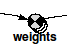Array-valued components

If a component's equation evaluates to an array, the component will be displayed with a repeated border as shown above, to indicate a stack of different values. An influence from that component to another will allow the array to be used in the destination component's equation in any of the ways described above. The number of layers in the stack indicates the outermost dimension of the array, up to a maximum of four. Only compartments, variables and flows can have array values.

There is only one way in which a list can appear in a model: by having an influence arrow coming out of a submodel with a variable number of instances. This means:

• a population submodel;
• a relation (association) submodel; or
• a conditional submodel.

As of Simile v6.1 an influence arrow within a special-purpose submodel can be set to deliver values from 'neighbour' components, which form a list as some instances have more neighbours than others.

## Processing lists

Lists can be used in functions and operators along with scalar values or other lists with the same member ids (e.g., from another variable in the same submodel). The rules for combining scalars and lists are similar to those for combining arrays as described above. Lists must be processed as soon as they appear in the variable outside the submodel which receives an influence arrow from a variable inside it. You cannot have a variable whose value is a list or array of lists. Rather, you must perform some legitimate list-processing operation, such as using the function sum(…) to sum the values of the list, so the variable's value is a scalar or fixed array.

As of Simile v6.1 the 'element()' function can be used to extract a sublist from a list, but the result is still a list, so some aggregating function must be applied to it when creating a component value from it.

In many cases, it is obvious how implicit replication will be applied when combining values with functions or operators. If one is scalar, then whatever the dimensions of the other, the result will have those dimensions. But what if the values are arrays with different nestings? If we add a 1-D array to a 2-D array, the result will be a 2-D array -- but how will the values of the 1-D array be shared out?

What happens is, the elements of the lesser-dimensional array are assigned to the elements of the higher-dimensional array with the same outer indices. For instance, [[1,2],[3,4]] + [10,20] is [[11,12], [23,24]] and not [[11,22], [13,24]]. Effectively the second argument is replicated from [10,20] to [[10,10], [20,20]]. Another way of looking at the same process is to imagine that the arguments are separated into groups, then the function applied to each group and the results combined back into arrays. So, [[1,2],[3,4]] + [10,20] becomes [ [1,2] + 10, [3,4] + 20 ].

The easiest way to remember how it is done is to learn the reason for it being that way, which is as follows: a modeller might wish to get either of the two possible results above, depending on the needs of their model. If they want the second case, they can be sure of getting it by using the makearray() function on the second argument, so they are actually adding [[1,2],[3,4]] and [[10,20], [10,20]] which have the same dimensions. However, if they want the first case, it would be more complicated to arrange it using such explicit replication, so to make things simpler, that is how implicit replication works.

# Dimensions

The dimensions field in the equation dialogue box is provided for reference only. It is not necessary to edit this information. The dimensions are a measure of the size of an array (or vector). Note that a vector is a one-dimensional array, and the term array is used here to cover both.

[2,3,4] has dimensions 3

[[2,3,4],[4,6,8]] has dimensions 2,3

# Physical units

## Unit definitions

Component values in Simile, and parameters used in equations, may be assigned physical units (e.g., cubic metres) by the modeller. There is a large set of such units built into Simile, and it can be extended with extra unit definitions provided by the modeller. The supplied units will be used to provide consistency checking and unit conversions where appropriate.

First off, there are the baseline units. These are the ones in terms of which all other units are defined. Simile comes with five baseline units predefined, metre (m), gramme (g), second (s), kelvin (k) and radian (rad). These are measures of length, mass, time, temperature and angle respectively. You can use either the short or long form to specify the units of a value.

Next there are the decimal multiplier prefixes, such as micro (). All those defined in SI are available. To use a decimally multiplied unit, simply put its prefix in short form before the short form of the unit itself, for instance kg = 1000 grammes (kilogramme). Some combinations of a multiplier and a base unit have a long form defined, e.g., kilogramme (kg), centimetre (cm). Again you can use either the short or long form to specify your units. Note that where appropriate we provide both the UK and US spellings.

Finally there are derived units, which are defined in terms of numerical constants and other units. You can write a derived unit using the short forms of other units (including other pre-defined derived units), integers and the symbols * (multiply), / (divide) and ^ (power). You can only use ^ with an integer exponent, as it works as a shorthand for multiplying, e.g., m^3 (cubic metre) means the same as m*m*m. There are also a number of pre-defined derived units. These include W (watt), psi (pound per square inch) and so forth. They may have a long form, and you can use either short or long form, and precede the short form with a decimal multiplier prefix, e.g., MJ for megajoule. Short forms of derived units can also be combined using symbols as above, e.g., W/m^2 = watts per square metre.

The definitions of all units are provided in the file new_units.pl in the Functions directory of the Simile installation. If you want to add extra units for your own application domain, create a file with a .pl extension in the appropriate place (see User-defined functions) and add the definitions in the same format as the built-in unit definitions. Note that all definitions define a short form in terms of other short forms -- you specify the longhands separately if they are needed. You cannot do anything with longhand forms except use them on their own to specify the units of model quantities -- all definitions, combinations and decimal multiplications must be done on short forms.

## Effects of setting units

There are two mechanisms within Simile for checking that physical units are compatible and for including a unit conversion where appropriate.

• Mechanism I is a method of using units to perform an ad-hoc conversion between values in different systems, without cluttering equations with conversion constants. This mechanism performs conversions between different Simile components.

• Mechanism II is a system of entering equations with dimension checking, enforcing consistency and performing the required conversions automatically. This mechanism performs conversions within a component's equation.

The first mechanism is always active. To activate the second mechanism, select the "Yes" option in the drop-down field labelled "Use units in math" in the Model...Properties dialogue box. The options are "Default", "Yes" or "No". The default is to inherit the setting from the parent submodel. In a top-level submodel (i.e. desktop), the default is "No". It is possible to have submodels with equation dimension checking and submodels without in the same model.

When you enter a new equation with the equation dialogue, you can enter a unit specification in the units field. If you do not do so, or if you use the equation bar, the value is given the default units for its equation. In the equation box, you can also specify units for the values associated with incoming influences. If you do not provide units for them, they get the same units as the component they come from.

### Mechanism I : Ad-hoc conversions

If you enter units for a value from an incoming influence, and the value of the component from which that influence comes also has units, Simile will perform a compatibility check and add a conversion if one is required. This makes it straightforward to include unit conversions when integrating models from different sources; provided the appropriate units are specified on each side of any link between them, the conversions will be built in automatically.

If the units at the source are real (i.e., it has no physical units) then it can be given any physical units at the destination and no conversion will be done. But if it does have physical units, then any units given to it at the destination must be compatible with them. That is to say they must have the same physical dimensions. For instance, mph (miles per hour) are compatible with m/s (metres per second) because they are both measures of speed. If one component has units of mph, and another has an influence from it which is given units of m/s, then the value from that influence that is used in the equation will be the result of converting the source value from mph to m/s, i.e., dividing it by about 2.25. However mph is not compatible with, say, km, because km is a measure of distance, not speed.

The same sort of check is also performed when a flow joins a compartment. Because the compartment's value is a function of the flow's value over time, the flow's units must be compatible with the units for the rate of change of the compartment over time. For instance it would be OK for the compartment to have units of litre and a connecting flow to have units of ft^3/day, because the first is volume and the second a rate of change for volume. In this case a conversion will be done on the amount of change due to the flow before adding it to or subtracting it from the compartment. But they could not both have units of litre, because the flow must be compatible with the compartment's rate of change rather than its actual value. In a future version of Simile we may allow a flow to have two different sorts of units at once, one for each end, to capture the situation in which it transforms one substance into another, e.g., a chemical reaction. In the mean time, if a flow has no physical units then no conversion will be performed at either end.

### Mechanism II : Fully consistent physical dimensions

When units checking within math is selected, setting an equation causes it to be checked for consistency of units. This works by generating a physical sort of units for each subexpression in the equation starting with the units of the incoming parameters, and ending with a default sort of units for the result.

When adding or subtracting two quantities, both quantities must have the same units. There is one exception: if one quantity is a numerical constant, it automatically takes the units of the other. If the two quantities have the same dimensions but do not also have the same units, it is necessary also to specify a conversion for one or both to the same units. This can be done by changing the units associated with a parameter as described in the last section. When that is done, a conversion will be applied to the parameter value before it is used in the equation, so it becomes compatible with the quantity added to or subtracted from it. For example, adding one distance in feet to another in metres, it is necessary first to either convert the former to metres, the latter to feet, or both to a third unit, such as km. The result will be in the units of both the arguments.

When multiplying or dividing two quantities, the units of the result are determined by multiplying or dividing the units of the quantities themselves. e.g., the result of dividing a parameter with units metre by one with units metre/second will have units second.

### Explicit conversion of dimensions

Although it should always be possible to create a model in which strict conservation of physical units applies, it is often the case that a model will include some ad-hoc representation of a complex relationship for which the exact equations are not available. In this case, the units of an equation's result may bear no relationship to those of its arguments. For instance, you might have a flow into a model compartment with dimensions mass/time, and the rate of this flow is set by a sketch graph against time. A sketch graph, like trig and exponential functions, must have a unitless argument, and produces a unitless result. However, the result of the time() function has dimensions time, and so cannot be used directly as the argument. For this kind of situation, you can include a unit name in the equation, with double-quotes around it to distinguish it from a parameter name, to represent unity with those units. So the argument for the graph function would be time()/"day" if the x-axis values on the graph represented numbers of days. Similarly, the result of the graph function is a unitless value, so it must be given units to allow it to make sense to the rest of the unit-checking-enabled model. This can be done by multiplying it by unit specifications -- for instance if the y-axis values on the graph represented mass transfer rates in kg/hour, the equation would be graph(...)*"kg"/"hour".  Of course the flow might go into a compartment whose units were some measure of mass other than kg, bit Simile would perform the appropriate conversions in this case.

If all the compatibility requirements are met, the end result of this process is a sort of units for the result of the equation. This is used as the default sort of units for the value being calculated. If you supply another sort of units for this value, it must be compatible with the default in terms of dimensions, and if the two are not the same an extra conversion may be applied after the equation has been calculated and before its value is assigned to the component. Note that the default units generated for the equation may not be in the most readable form, for instance if a result in watts is generated the units from the components might actually be something like N*m/s. In such a case you can set the units manually to the normal form, and no conversion will be done because they are mathematically equivalent.

In the physical sciences, many models can be completely expressed in terms of physically meaningful quantities. With this sort of model, it is a good idea to enable units checking within math, as it provides an extra safeguard against mistakes. In ecology and the social sciences, it is more common to use ad-hoc parameterisation that defies physical interpretation. In these cases, it is probably not useful to use this system, which is why it is switched off by default.

# Enumerated types

Enumerated types allow you to refer to a each object in a collection by name, where the alternative is to use an arbitrary index number. To understand what is meant by an arbitrary index number, consider the following contrasting situations.

Let's say, for example, that you want to model soil water dynamics, with the soil divided into (say) four layers. You would create a submodel with four instances, numbered 1 to 4. You can then find the value for water content in the third layer using an expression such as element([water],3). In this case, the index number refers meaningfully to the layer number.

Now, suppose you wanted to model the growth and yield of four fruit trees: say apples, oranges, lemons and pears. You could use the above approach, creating a submodel with four instances, but in this case you would have to remember that instance 1 is "apples", 2 is "oranges", and so on. The index number is now an arbitrary index number.

The enumerated type mechanism enables you to associate the name of each fruit with each instance of the submodel, and to use this name (rather than a number) when referring to a particular instance. This considerably increases the clarity of your model, since someone trying to follow the logic of the model does not need to keep on checking up on the correspondence between an integer number and some more meaningful label.

## Defining an enumerated type

Before you can use an enumerated type, you must define it. Enumerated types are defined at the level of the submodel. All submodels contained within the submodel in which the type is defined also have access to the definition. It is therefore most simple to define it for the whole model (so that any submodel in the model can then use it). To do this:

1. Go into the submodel properties dialogue for the Desktop, either by doubleclicking on the background or by selecting "Properties..." from the edit or context menus
2. Click on the "Advanced" tab in the Properties dialogue window to view the advanced options.
3. Click in the edit field above the "Add type" button, type in the name for the enumerated type, e.g. "fruit", and click the "Add type" button.
4. Click on the word "fruit" now entered into the Enumerated types list, to highlight it.
5. Type "apples" into the same edit field, and click on "Add member".
6. Repeat for "oranges", "lemons" and "pears". If at any stage the word "fruit" becomes un-selected, select it again to enable you to add new members.
7. To see what the list of values is for "fruit", hover over the name and observe the pop-up reminder.
8. You can now (or at some later stage) define additional enumerated types in the same way.

Alternatively, once you have supplied the name of the type, you can read the members from a file. To do this, click on the "Get from file" button. This displays the table entry dialogue, and you can use this as if you were specifying a data table to load. However, the data is not actually loaded; instead, all the different values are listed, removing duplicates, and these are made into the members of your enumerated type. The values must be textual, not numbers, so only data entry from columns or grids is allowed.

## Using an enumerated type

The following model diagram will serve to illustrate how an enumerated type can be used. It shows a variable size inside a multiple-instance submodel (one instance for each fruit). This variable is exported as an array outside the submodel, then one value is picked up from this array.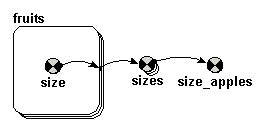If this model were set up without using an enumerated type, then the following would be used:

Submodel fruit: Number of instances (using specified dimensions): 4

Variable size: if index(1)==1 then 4.2 else 3.7

Variable sizes: [size] (Dimensions are automatically set to 4)

Variable size_apples: element([sizes],1)

If this model (with the same model diagram) were set up using an enumerated type (e.g. fruit, as defined above), then the following would be used:

Submodel fruit: Number of instances (using specified dimensions): fruit (i.e. the name of the enumerated type is entered into the dimensions edit field, and automatically sets the number of instances for the submodel to the number of values in the enumerated type - in this case, four.)

Variable size: if index(1)=="apples" then 4.2 else 3.7

Variable sizes: [size] (dimensions are automatically set to fruit)

Variable size_apples: element([sizes],"apples")

### Things to note:

The same enumerated type can be used in several places. For example, you could have another submodel that also has dimensions of fruit.

Note that the only test we can do with an enumerated type value is a test of equality with the index of a submodel (if index(1)=="apples"...). We can't use the greater-than or less-than operators, since the list is unordered (what a statistician would call nominal rather than ordinal).

You can use the member names as indices when reading parameter values from a file. This is particularly convenient when the file was used to create the list of member names.

For example, the following file could be used

fruit, price

apples, 7.0

oranges, 7.4

lemons, 7.2

pears, 8.1

### Using array functions

You can use the makearray function to create an array. For example, the equation

makearray(1,"fruit")

will create an array with dimensions (size) equal to the number of members in the enumerated type "fruit". It will be populated with values of 1. You can use the test place_in(n)==member to set each value separately. For example, the equation

makearray(if place_in(1)=="apples" then 7.0 elseif place_in(1)=="oranges" then 7.4 elseif place_in(1)=="lemons" then 7.2 else 8.1, "fruit")

will result in the same array as the above table.

### Why is it called an enumerated type?

In programming, a type is a set of values from which a variable may take its value. Thus if a variable is of type "integer", then this set of values is all the whole numbers. If it is of type "real", then it is the set of all decimal values that can be represented on the computer. If it is an enumerated type, then the set of values is defined by an explicit list of all the values that the variable is allowed to take. The standard example is the enumerated type "days of the week", in which the set of allowed values is given by the list [Monday, Tuesday, Wednesday, Thursday, Friday, Saturday, Sunday]. A particular variable (e.g. "washing day") can be defined to be of this type: we then know that its value must be one of these seven possible values.

# Local names

The local name is automatically composed from the full path name by replacing characters that are illegal in equations (such as spaces and carriage returns) with underscores. If you would prefer to use a different local name to refer to the full path name, the local name can be edited. To do this, you must have the equation dialogue box open, and click on the tab labeled "Parameters etc.".

Right-click on the name in the list box to edit it. Each other model element can have only one local name in this one (though the other model element might be referred to using a different local name in further other model elements expressions). For this reason, if you edit the local name, you may also need to edit the expression itself to update the corresponding references.

# Working with equations : Equation Listings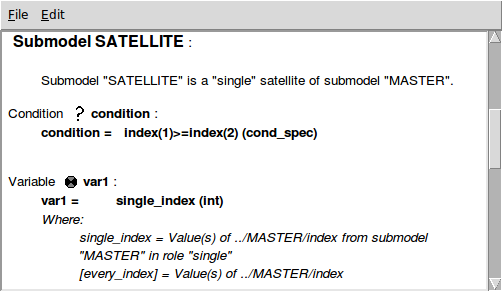For documentation purposes, it may be necessary to produce a listing of all equations in a model, including such information as how the value of each other component is referred to in the equation, the definitions of any enumerated types used, and so forth. This can be done by selecting the 'List equations' entry from the 'Model' menu. The listing can be selected and copied from its window, and pasted into other applications.